# Aggregating Data with dplyr VIDEO

Aggregating data is a critical step in the data analysis process. In the video below, you will see an example of how to aggregate data using the dplyr package from R.# More Selecting and Transforming with dplyR

In this post, we are going to learn some more advance ways to work with functions in the dplyr package. Let’s load our libraries

``````library(dplyr)
library(gapminder)``````

Our dataset is the gapminder dataset which provides information about countries and continents related to gdp, life expectancy, and population. Here is what the data looks like as a refresher.

``glimpse(gapminder)``
``````## Rows: 1,704
## Columns: 6
## \$ country   <fct> "Afghanistan", "Afghanistan", "Afghanistan", "Afghanistan", …
## \$ continent <fct> Asia, Asia, Asia, Asia, Asia, Asia, Asia, Asia, Asia, Asia, …
## \$ year      <int> 1952, 1957, 1962, 1967, 1972, 1977, 1982, 1987, 1992, 1997, …
## \$ lifeExp   <dbl> 28.801, 30.332, 31.997, 34.020, 36.088, 38.438, 39.854, 40.8…
## \$ pop       <int> 8425333, 9240934, 10267083, 11537966, 13079460, 14880372, 12…
## \$ gdpPercap <dbl> 779.4453, 820.8530, 853.1007, 836.1971, 739.9811, 786.1134, …``````

# select

You can use the colon symbol to select multiple columns at once. Doing this is a great way to save time when selecting variables.

``````gapminder%>%
select(lifeExp:gdpPercap)``````
``````## # A tibble: 1,704 x 3
##    lifeExp      pop gdpPercap
##      <dbl>    <int>     <dbl>
##  1    28.8  8425333      779.
##  2    30.3  9240934      821.
##  3    32.0 10267083      853.
##  4    34.0 11537966      836.
##  5    36.1 13079460      740.
##  6    38.4 14880372      786.
##  7    39.9 12881816      978.
##  8    40.8 13867957      852.
##  9    41.7 16317921      649.
## 10    41.8 22227415      635.
## # … with 1,694 more rows``````

You can see that by using the colon we were able to select the last three columns.

There are also arguments called “select helpers.” Select helpers help you find columns in really large data sets. For example, let’s say we want columns that contain the string “life” in them. To find this we would use the contain argument as shown below.

``````gapminder%>%
select(contains('life'))``````
``````## # A tibble: 1,704 x 1
##    lifeExp
##      <dbl>
##  1    28.8
##  2    30.3
##  3    32.0
##  4    34.0
##  5    36.1
##  6    38.4
##  7    39.9
##  8    40.8
##  9    41.7
## 10    41.8
## # … with 1,694 more rows``````

Only the column that contains the string life is selected. There are other help selectors that you can try on your own such as starts_with, ends_with and more.

To remove a variable from a dataset you simply need to put a minus sign in front of it as shown below.

``````gapminder %>%
select(-lifeExp, -gdpPercap)``````
``````## # A tibble: 1,704 x 4
##    country     continent  year      pop
##    <fct>       <fct>     <int>    <int>
##  1 Afghanistan Asia       1952  8425333
##  2 Afghanistan Asia       1957  9240934
##  3 Afghanistan Asia       1962 10267083
##  4 Afghanistan Asia       1967 11537966
##  5 Afghanistan Asia       1972 13079460
##  6 Afghanistan Asia       1977 14880372
##  7 Afghanistan Asia       1982 12881816
##  8 Afghanistan Asia       1987 13867957
##  9 Afghanistan Asia       1992 16317921
## 10 Afghanistan Asia       1997 22227415
## # … with 1,694 more rows``````

In the output above you can see that life expectancy and per capa GDP are missing.

# rename

Another function is the rename function which allows you to rename a variable. Below is an example in which the variable “pop” is renamed “population.”

``````gapminder %>%
select(country, year, pop) %>%
rename(population=pop)``````
``````## # A tibble: 1,704 x 3
##    country      year population
##    <fct>       <int>      <int>
##  1 Afghanistan  1952    8425333
##  2 Afghanistan  1957    9240934
##  3 Afghanistan  1962   10267083
##  4 Afghanistan  1967   11537966
##  5 Afghanistan  1972   13079460
##  6 Afghanistan  1977   14880372
##  7 Afghanistan  1982   12881816
##  8 Afghanistan  1987   13867957
##  9 Afghanistan  1992   16317921
## 10 Afghanistan  1997   22227415
## # … with 1,694 more rows``````

You can see that the “pop” variable has been renamed. Remember that the new name goes on the left of the equal sign while the old name goes on the right of the equal sign.

There is a shortcut to this and it involves renaming variables inside the select function. In the example below, we rename the pop variable population inside the select function.

``````gapminder %>%
select(country, year, population=pop)``````
``````## # A tibble: 1,704 x 3
##    country      year population
##    <fct>       <int>      <int>
##  1 Afghanistan  1952    8425333
##  2 Afghanistan  1957    9240934
##  3 Afghanistan  1962   10267083
##  4 Afghanistan  1967   11537966
##  5 Afghanistan  1972   13079460
##  6 Afghanistan  1977   14880372
##  7 Afghanistan  1982   12881816
##  8 Afghanistan  1987   13867957
##  9 Afghanistan  1992   16317921
## 10 Afghanistan  1997   22227415
## # … with 1,694 more rows``````

# transmute

The transmute function allows you to select and mutate variables at the same time. For example, let’s say that we want to know total gdp we could find this by multplying the population by gdp per capa. This is done with the transmute function below.

``````gapminder %>%
transmute(country, year, total_gdp = pop * gdpPercap)``````
``````## # A tibble: 1,704 x 3
##    country      year    total_gdp
##    <fct>       <int>        <dbl>
##  1 Afghanistan  1952  6567086330.
##  2 Afghanistan  1957  7585448670.
##  3 Afghanistan  1962  8758855797.
##  4 Afghanistan  1967  9648014150.
##  5 Afghanistan  1972  9678553274.
##  6 Afghanistan  1977 11697659231.
##  7 Afghanistan  1982 12598563401.
##  8 Afghanistan  1987 11820990309.
##  9 Afghanistan  1992 10595901589.
## 10 Afghanistan  1997 14121995875.
## # … with 1,694 more rows``````

Conclusion

With these basic tools it is now a little easier to do some data analysis when using R. There is so much more than can be learned but this will have to wait for the future.

# Transform Variables with dplyR VIDEO

Handling data is a key skill for a data analyst. In the video below examples are provided of how to transform variables using functions in the dplyr package.# Data Aggregation with dplyr

In this post, we will learn about data aggregation with the dplyr package. Data aggregation is primarily a tool for summarizing the information you have collected. Let’s start by loading our packages.

``````library(dplyr)
library(gapminder)``````

dplyr is for the data manipulation while gapminder provides us with the data. We will learn the following functions from the dplyr package

• count()
• summarize
• group_by()
• top_n()

# count

The count function allows you to count the number of observations in your dataset as shown below.

``````gapminder %>%
count()``````
``````## # A tibble: 1 x 1
##       n
##   <int>
## 1  1704``````

The output tells us that there are over 1700 rows of data. However, the count function can do much more. For example, we can also count values in a specific column. Below, we calculated how many rows of data we have by continent.

``````gapminder %>%
count(continent)``````
``````## # A tibble: 5 x 2
##   continent     n
##   <fct>     <int>
## 1 Africa      624
## 2 Americas    300
## 3 Asia        396
## 4 Europe      360
## 5 Oceania      24``````

The output speaks for its self. There are two columns the left is continent and the right is how many times that particular continent appears in the dataset. You can also sort this data by adding the argument called sort as shown below.

``````gapminder %>%
count(continent, sort =TRUE)``````
``````## # A tibble: 5 x 2
##   continent     n
##   <fct>     <int>
## 1 Africa      624
## 2 Asia        396
## 3 Europe      360
## 4 Americas    300
## 5 Oceania      24``````

There is another argument we can add and this is called the weight or wt argument. The wt argument adds up the values of the population in our example and we can now see how many respondents there were from each continent. Below is the code an example

``````gapminder %>%
count(continent, wt=pop, sort=TRUE)``````
``````## # A tibble: 5 x 2
##   continent           n
##   <fct>           <dbl>
## 1 Asia      30507333901
## 2 Americas   7351438499
## 3 Africa     6187585961
## 4 Europe     6181115304
## 5 Oceania     212992136``````

You can see that we now know how many people from each continent were in the dataset.

# summarize

The summarize function takes many rows of data and reduce it to a single output. For example, if we want to know the total number of people in the dataset we could run the code below.

``````gapminder %>%
summarize(total_pop=sum(pop))``````
``````## # A tibble: 1 x 1
##     total_pop
##         <dbl>
## 1 50440465801``````

You can also continue to add more and more things you want to know be separating them with a comma. In the code below, we add to it the average GDP.

``````gapminder %>%
summarize(total_pop=sum(pop), average_gdp=mean(gdpPercap))``````
``````## # A tibble: 1 x 2
##     total_pop average_gdp
##         <dbl>       <dbl>
## 1 50440465801       7215.``````

# group_by

The group by function allows you to aggregate data by groups. For example, if we want to know the total population and the average gdp by continent the code below would help to learn this.

``````gapminder %>%
group_by(continent) %>%
summarize(total_pop=sum(pop), mean_gdp=mean(gdpPercap)) %>%
arrange(desc(total_pop))``````
``````## # A tibble: 5 x 3
##   continent   total_pop mean_gdp
##   <fct>           <dbl>    <dbl>
## 1 Asia      30507333901    7902.
## 2 Americas   7351438499    7136.
## 3 Africa     6187585961    2194.
## 4 Europe     6181115304   14469.
## 5 Oceania     212992136   18622.``````

It is also possible to group by more than one column. However, to do this we need to create another categorical variable. We are going to use mutate to create a categorical variable that breaks the data into two parts. Before 1980 and after 1980. Then we will group by country and whether the mean of the gdp was collected before or after 1980. Below is the code

``````gapminder %>%
mutate(before_1980=if_else(year < 1980, "yes","no")) %>%
group_by(country, before_1980) %>%
summarize(mean_gdp=mean(gdpPercap))``````
``````## # A tibble: 284 x 3
## # Groups:   country 
##    country     before_1980 mean_gdp
##    <fct>       <chr>          <dbl>
##  1 Afghanistan no              803.
##  2 Afghanistan yes             803.
##  3 Albania     no             3934.
##  4 Albania     yes            2577.
##  5 Algeria     no             5460.
##  6 Algeria     yes            3392.
##  7 Angola      no             2944.
##  8 Angola      yes            4270.
##  9 Argentina   no             9998.
## 10 Argentina   yes            7913.
## # … with 274 more rows``````

# top_n

The top_n function allows you to find the most extreme values when looking at groups. For example, we could find which countries has the highest life expectancy by continent. The answer is below

``````gapminder %>%
group_by(continent) %>%
top_n(1, lifeExp)``````
``````## # A tibble: 5 x 6
## # Groups:   continent 
##   country   continent  year lifeExp       pop gdpPercap
##   <fct>     <fct>     <int>   <dbl>     <int>     <dbl>
## 1 Australia Oceania    2007    81.2  20434176    34435.
## 2 Canada    Americas   2007    80.7  33390141    36319.
## 3 Iceland   Europe     2007    81.8    301931    36181.
## 4 Japan     Asia       2007    82.6 127467972    31656.
## 5 Reunion   Africa     2007    76.4    798094     7670.``````

As an example, Japan has the highest life expectancy in Asia. Canada has the highest life expectancy in the Americas. Naturally you are not limited to the top 1. This number can be changed to whatever you want. For example, below we change the number to 3.

``````gapminder %>%
group_by(continent) %>%
top_n(3, lifeExp)``````
``````## # A tibble: 15 x 6
## # Groups:   continent 
##    country          continent  year lifeExp       pop gdpPercap
##    <fct>            <fct>     <int>   <dbl>     <int>     <dbl>
##  1 Australia        Oceania    2002    80.4  19546792    30688.
##  2 Australia        Oceania    2007    81.2  20434176    34435.
##  3 Canada           Americas   2002    79.8  31902268    33329.
##  4 Canada           Americas   2007    80.7  33390141    36319.
##  5 Costa Rica       Americas   2007    78.8   4133884     9645.
##  6 Hong Kong, China Asia       2007    82.2   6980412    39725.
##  7 Iceland          Europe     2007    81.8    301931    36181.
##  8 Japan            Asia       2002    82   127065841    28605.
##  9 Japan            Asia       2007    82.6 127467972    31656.
## 10 New Zealand      Oceania    2007    80.2   4115771    25185.
## 11 Reunion          Africa     1997    74.8    684810     6072.
## 12 Reunion          Africa     2002    75.7    743981     6316.
## 13 Reunion          Africa     2007    76.4    798094     7670.
## 14 Spain            Europe     2007    80.9  40448191    28821.
## 15 Switzerland      Europe     2007    81.7   7554661    37506.``````# Transform Data with dplyr

In this post, we will be exposed to tools for wrangling and manipulating data in R.

Let’s begin by loading the libraries we will be using. We will use the dplyr package and the gapminder package. dplyr is for manipulating the data and gapminder provides the dataset.

``````library(dplyr)
library(gapminder)``````

You can look at the data briefly by using a function called “glimpse” as shown below.

``glimpse(gapminder)``
``````## Rows: 1,704
## Columns: 6
## \$ country   <fct> "Afghanistan", "Afghanistan", "Afghanistan", "Afghanistan", …
## \$ continent <fct> Asia, Asia, Asia, Asia, Asia, Asia, Asia, Asia, Asia, Asia, …
## \$ year      <int> 1952, 1957, 1962, 1967, 1972, 1977, 1982, 1987, 1992, 1997, …
## \$ lifeExp   <dbl> 28.801, 30.332, 31.997, 34.020, 36.088, 38.438, 39.854, 40.8…
## \$ pop       <int> 8425333, 9240934, 10267083, 11537966, 13079460, 14880372, 12…
## \$ gdpPercap <dbl> 779.4453, 820.8530, 853.1007, 836.1971, 739.9811, 786.1134, …``````

You can see that we have six columns or variables and over 1700 rows of data. This data provides information about countries and various demographic statistics.

# select()

The select function allows you to grab only the variables you want for analysis. This becomes exceptionally important when you have a large number of variables. In our next example, we will select 4 variables from the gapminder dataset. Below is the code to achieve this.

``````gapminder %>%
select(country,continent, pop, lifeExp)``````
``````## # A tibble: 1,704 x 4
##    country     continent      pop lifeExp
##    <fct>       <fct>        <int>   <dbl>
##  1 Afghanistan Asia       8425333    28.8
##  2 Afghanistan Asia       9240934    30.3
##  3 Afghanistan Asia      10267083    32.0
##  4 Afghanistan Asia      11537966    34.0
##  5 Afghanistan Asia      13079460    36.1
##  6 Afghanistan Asia      14880372    38.4
##  7 Afghanistan Asia      12881816    39.9
##  8 Afghanistan Asia      13867957    40.8
##  9 Afghanistan Asia      16317921    41.7
## 10 Afghanistan Asia      22227415    41.8
## # … with 1,694 more rows``````

The strange symbol %>% is called a “pipe” and allows you to continuously build your code. You can also save this information by assigning a name to an object like any other variable in r.

``````country_data<-gapminder %>%
select(country,continent, pop, lifeExp)``````

# arrange

The arrange verb sorts your data based on one or more variables. For example, let’s say we want to know which country has the highest population. The code below provides the answer.

``````country_data %>%
arrange(pop)``````
``````## # A tibble: 1,704 x 4
##    country               continent   pop lifeExp
##    <fct>                 <fct>     <int>   <dbl>
##  1 Sao Tome and Principe Africa    60011    46.5
##  2 Sao Tome and Principe Africa    61325    48.9
##  3 Djibouti              Africa    63149    34.8
##  4 Sao Tome and Principe Africa    65345    51.9
##  5 Sao Tome and Principe Africa    70787    54.4
##  6 Djibouti              Africa    71851    37.3
##  7 Sao Tome and Principe Africa    76595    56.5
##  8 Sao Tome and Principe Africa    86796    58.6
##  9 Djibouti              Africa    89898    39.7
## 10 Sao Tome and Principe Africa    98593    60.4
## # … with 1,694 more rows``````

To complete this task we had to use the arrange function and place the name of the variable we want to sort by inside the parentheses. However, this is not exactly what we want. What we have found is the countries with the smallest population. To sort from largest to smallest you must use the desc function as well and this is shown below.

``````country_data %>%
arrange(desc(pop))``````
``````## # A tibble: 1,704 x 4
##    country continent        pop lifeExp
##    <fct>   <fct>          <int>   <dbl>
##  1 China   Asia      1318683096    73.0
##  2 China   Asia      1280400000    72.0
##  3 China   Asia      1230075000    70.4
##  4 China   Asia      1164970000    68.7
##  5 India   Asia      1110396331    64.7
##  6 China   Asia      1084035000    67.3
##  7 India   Asia      1034172547    62.9
##  8 China   Asia      1000281000    65.5
##  9 India   Asia       959000000    61.8
## 10 China   Asia       943455000    64.0
## # … with 1,694 more rows``````

Now, this is what we want. China claims several of the top spots. The reason a country is on the list more than once is that the data was collected several different years.

# filter

The filter function is used to obtain only specific values that meet the criteria. For example, what if we want to know the population of only India in descending order. Below is the code for how to do this.

``````country_data %>%
arrange(desc(pop)) %>%
filter(country=='India')        ``````
``````## # A tibble: 12 x 4
##    country continent        pop lifeExp
##    <fct>   <fct>          <int>   <dbl>
##  1 India   Asia      1110396331    64.7
##  2 India   Asia      1034172547    62.9
##  3 India   Asia       959000000    61.8
##  4 India   Asia       872000000    60.2
##  5 India   Asia       788000000    58.6
##  6 India   Asia       708000000    56.6
##  7 India   Asia       634000000    54.2
##  8 India   Asia       567000000    50.7
##  9 India   Asia       506000000    47.2
## 10 India   Asia       454000000    43.6
## 11 India   Asia       409000000    40.2
## 12 India   Asia       372000000    37.4``````

Now we have only data that relates to India. All we did was include one more pipe and the filter function. We had to tell R which country by placing the information above in the parentheses.

filter is not limited to text searches. You can also search based on numerical values. For example, what if we only want countries with a life expectancy of 81 or higher

``````country_data %>%
arrange(desc(pop)) %>%
filter(lifeExp >= 81)``````
``````## # A tibble: 7 x 4
##   country          continent       pop lifeExp
##   <fct>            <fct>         <int>   <dbl>
## 1 Japan            Asia      127467972    82.6
## 2 Japan            Asia      127065841    82
## 3 Australia        Oceania    20434176    81.2
## 4 Switzerland      Europe      7554661    81.7
## 5 Hong Kong, China Asia        6980412    82.2
## 6 Hong Kong, China Asia        6762476    81.5
## 7 Iceland          Europe       301931    81.8``````

You can see the results for yourself. It is also possible to combine multiply conditions for whatever functions are involved. For example, if I want to arrange my data by population and country while also filtering it by a population greater than 100,000,000,000 and with a life expectancy of less than 45. This is shown below

``````country_data %>%
arrange(desc(pop, country)) %>%
filter(pop>100000000, lifeExp<45)``````
``````## # A tibble: 5 x 4
##   country continent       pop lifeExp
##   <fct>   <fct>         <int>   <dbl>
## 1 China   Asia      665770000    44.5
## 2 China   Asia      556263527    44
## 3 India   Asia      454000000    43.6
## 4 India   Asia      409000000    40.2
## 5 India   Asia      372000000    37.4``````

# mutate

The mutate function is for manipulating variables and creating new ones. For example, the gdpPercap variable is highly skewed. We can create a variable of gdpercap that is the log of this variable. Using the log will help the data to assume the characteristics of a normal distribution. Below is the code for this.

``````gapminder %>%
select(country,continent, pop, gdpPercap) %>%
mutate(log_gdp=log(gdpPercap))``````
``````## # A tibble: 1,704 x 5
##    country     continent      pop gdpPercap log_gdp
##    <fct>       <fct>        <int>     <dbl>   <dbl>
##  1 Afghanistan Asia       8425333      779.    6.66
##  2 Afghanistan Asia       9240934      821.    6.71
##  3 Afghanistan Asia      10267083      853.    6.75
##  4 Afghanistan Asia      11537966      836.    6.73
##  5 Afghanistan Asia      13079460      740.    6.61
##  6 Afghanistan Asia      14880372      786.    6.67
##  7 Afghanistan Asia      12881816      978.    6.89
##  8 Afghanistan Asia      13867957      852.    6.75
##  9 Afghanistan Asia      16317921      649.    6.48
## 10 Afghanistan Asia      22227415      635.    6.45
## # … with 1,694 more rows``````

In the code above we had to select our variables again and then we create the new variable “log_gdp”. This new variable appears all the way to the right in the dataset. Naturally, we can extend our code by using our new variable in other functions as shown below.

Conclusion

This post was longer than normal but several practical things were learned. You now know some basic techniques for wrangling data using the dplyr package in R.

# Principal Component Analysis with Python VIDEO

Principal component analysis is a tool for reducing the number of variables in a dataset without losing too much information. This is a great way to summarize information or to simplify things for a more complex analysis. The video provides a simple example of how to do this.# T-SNE Visualization and R

It is common in research to want to visualize data in order to search for patterns. When the number of features increases, this can often become even more important. Common tools for visualizing numerous features include principal component analysis and linear discriminant analysis. Not only do these tools work for visualization they can also be beneficial in dimension reduction.However, the available tools for us are not limited to these two options. Another option for achieving either of these goals is t-Distributed Stochastic Embedding. This relative young algorithm (2008) is the focus of the post. We will explain what it is and provide an example using a simple dataset from the Ecdat package in R.

## t-sne Defined

t-sne is a nonlinear dimension reduction visualization tool. Essentially what it does is identify observed clusters. However, it is not a clustering algorithm because it reduces the dimensions (normally to 2) for visualizing. This means that the input features are not longer present in their original form and this limits the ability to make inference. Therefore, t-sne is often used for exploratory purposes.

T-sne non-linear characteristic is what makes it often appear to be superior to PCA, which is linear. Without getting too technical t-sne takes simultaneously a global and local approach to mapping points while PCA can only use a global approach.

The downside to t-sne approach is that it requires a large amount of calculations. The calculations are often pairwise comparisons which can grow exponential in large datasets.

## Initial Packages

We will use the “Rtsne” package for the analysis, and we will use the “Fair” dataset from the “Ecdat” package. The “Fair” dataset is data collected from people who had cheated on their spouse. We want to see if we can find patterns among the unfaithful people based on their occupation. Below is some initial code.

``````library(Rtsne)
library(Ecdat)``````

## Dataset Preparation

To prepare the data, we first remove in rows with missing data using the “na.omit” function. This is saved in a new object called “train”. Next, we change or outcome variable into a factor variable. The categories range from 1 to 9

1. Farm laborer, day laborer,
2. Unskilled worker, service worker,
3. Machine operator, semiskilled worker,
4. Skilled manual worker, craftsman, police,
5. Clerical/sales, small farm owner,
6. Technician, semiprofessional, supervisor,
7. Small business owner, farm owner, teacher,
8. Mid-level manager or professional,
9. Senior manager or professional.

Below is the code.

``````train<-na.omit(Fair)
train\$occupation<-as.factor(train\$occupation)``````

## Visualization Preparation

Before we do the analysis we need to set the colors for the different categories. This is done with the code below.

``````colors<-rainbow(length(unique(train\$occupation)))
names(colors)<-unique(train\$occupation)``````

We can now do are analysis. We will use the “Rtsne” function. When you input the dataset you must exclude the dependent variable as well as any other factor variables. You also set the dimensions and the perplexity. Perplexity determines how many neighbors are used to determine the location of the datapoint after the calculations. Verbose just provides information during the calculation. This is useful if you want to know what progress is being made. max_iter is the number of iterations to take to complete the analysis and check_duplicates checks for duplicates which could be a problem in the analysis. Below is the code.

``tsne<-Rtsne(train[,-c(1,4,7)],dims=2,perplexity=30,verbose=T,max_iter=1500,check_duplicates=F)``
``````## Performing PCA
## Read the 601 x 6 data matrix successfully!
## OpenMP is working. 1 threads.
## Using no_dims = 2, perplexity = 30.000000, and theta = 0.500000
## Computing input similarities...
## Building tree...
## Done in 0.05 seconds (sparsity = 0.190597)!
## Learning embedding...
## Iteration 1450: error is 0.280471 (50 iterations in 0.07 seconds)
## Iteration 1500: error is 0.279962 (50 iterations in 0.07 seconds)
## Fitting performed in 2.21 seconds.``````

Below is the code for making the visual.

``````plot(tsne\$Y,t='n',main='tsne',xlim=c(-30,30),ylim=c(-30,30))
text(tsne\$Y,labels=train\$occupation,col = colors[train\$occupation])
legend(25,5,legend=unique(train\$occupation),col = colors,,pch=c(1))``````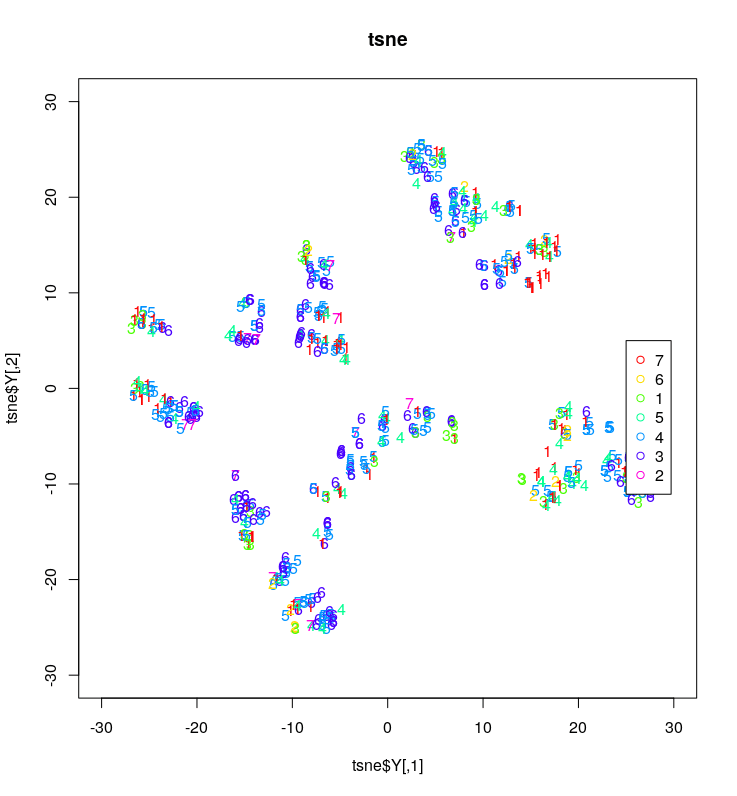You can see that there are clusters however, the clusters are all mixed with the different occupations. What this indicates is that the features we used to make the two dimensions do not discriminant between the different occupations.

Conclusion

T-SNE is an improved way to visualize data. This is not to say that there is no place for PCA anymore. Rather, this newer approach provides a different way of quickly visualizing complex data without the limitations of PCA.

Checkout ERT online courses# Quadratic Discriminant Analysis with Python

Quadratic discriminant analysis allows for the classifier to assess non -linear relationships. This of course something that linear discriminant analysis is not able to do. This post will go through the steps necessary to complete a qda analysis using Python. The steps that will be conducted are as follows1. Data preparation
2. Model training
3. Model testing

Our goal will be to predict the gender of examples in the “Wages1” dataset using the available independent variables.

Data Preparation

```import pandas as pd
from pydataset import data
import matplotlib.pyplot as plt
from sklearn.discriminant_analysis import QuadraticDiscriminantAnalysis as QDA
from sklearn.model_selection import train_test_split
from sklearn.metrics import classification_report
from sklearn.metrics import (confusion_matrix,accuracy_score)
import seaborn as sns
from matplotlib.colors import ListedColormap```

Next, we will load our data “Wages1” it comes from the “pydataset” library. After loading the data, we will use the .head() method to look at it briefly.We need to transform the variable ‘sex’, our dependent variable, into a dummy variable using numbers instead of text. We will use the .getdummies() method to make the dummy variables and then add them to the dataset using the .concat() method. The code for this is below.

In the code below we have the histogram for the continuous independent variables.  We are using the .distplot() method from seaborn to make the histograms.

```fig = plt.figure()
fig, axs = plt.subplots(figsize=(15, 10),ncols=3)
sns.set(font_scale=1.4)
sns.distplot(df['exper'],color='black',ax=axs)
sns.distplot(df['school'],color='black',ax=axs)
sns.distplot(df['wage'],color='black',ax=axs)```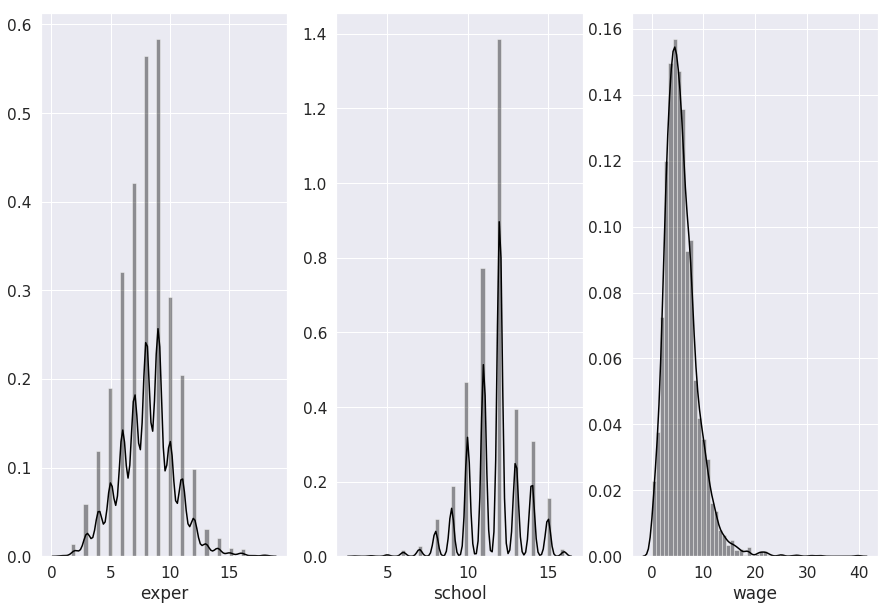The variables look reasonable normal. Below is the proportions of the categorical dependent variable.

```round(df.groupby('sex').count()/3294,2)
Out:
exper school wage female male
sex
female 0.48 0.48 0.48 0.48 0.48
male 0.52 0.52 0.52 0.52 0.52```

About half male and half female.

We will now make the correlational matrix

```corrmat=df.corr(method='pearson')
f,ax=plt.subplots(figsize=(12,12))
sns.set(font_scale=1.2)
sns.heatmap(round(corrmat,2),
vmax=1.,square=True,
cmap="gist_gray",annot=True)```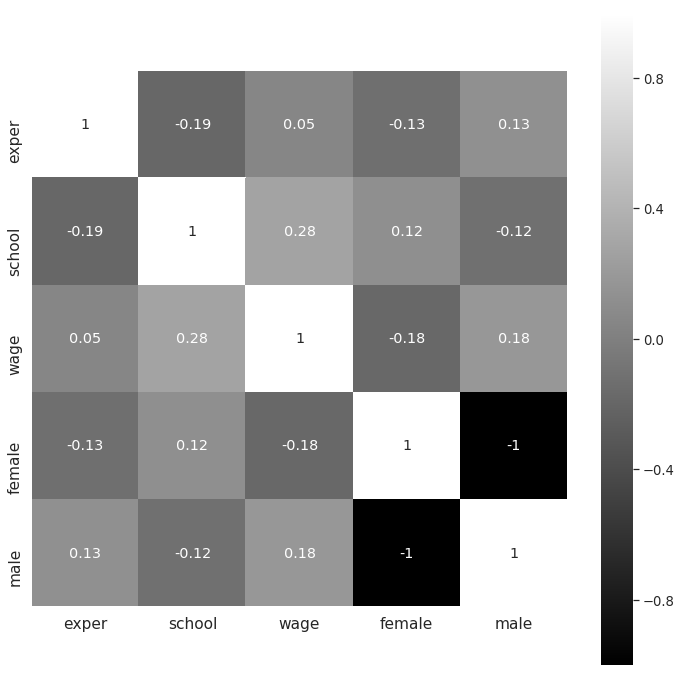There appears to be no major problems with correlations. The last thing we will do is set up our train and test datasets.

```X=df[['exper','school','wage']]
y=df['male']
X_train,X_test,y_train,y_test=train_test_split(X,y,
test_size=.2, random_state=50)```

We can now move to model development

Model Development

To create our model we will instantiate an instance of the quadratic discriminant analysis function and use the .fit() method.

```qda_model=QDA()
qda_model.fit(X_train,y_train)```

There are some descriptive statistics that we can pull from our model. For our purposes, we will look at the group means  Below are the  group means.

 exper school wage Female 7.73 11.84 5.14 Male 8.28 11.49 6.38

You can see from the table that mean generally have more experience, higher wages, but slightly less education.

We will now use the qda_model we create to predict the classifications for the training set. This information will be used to make a confusion matrix.

```cm = confusion_matrix(y_train, y_pred)
ax= plt.subplots(figsize=(10,10))
sns.set(font_scale=3.4)
with sns.axes_style('white'):
sns.heatmap(cm, cbar=False, square=True, annot=True, fmt='g',
cmap=ListedColormap(['gray']), linewidths=2.5)```The information in the upper-left corner are the number of people who were female and correctly classified as female. The lower-right corner is for the men who were correctly classified as men. The upper-right corner is females who were classified as male. Lastly, the lower-left corner is males who were classified as females. Below is the actually accuracy of our model

```round(accuracy_score(y_train, y_pred),2)
Out: 0.6```

Sixty percent accuracy is not that great. However, we will now move to model testing.

Model Testing

Model testing involves using the .predict() method again but this time with the testing data. Below is the prediction with the confusion matrix.

``` y_pred=qda_model.predict(X_test)
cm = confusion_matrix(y_test, y_pred)
from matplotlib.colors import ListedColormap
ax= plt.subplots(figsize=(10,10))
sns.set(font_scale=3.4)
with sns.axes_style('white'):
sns.heatmap(cm, cbar=False, square=True,annot=True,fmt='g',
cmap=ListedColormap(['gray']),linewidths=2.5)```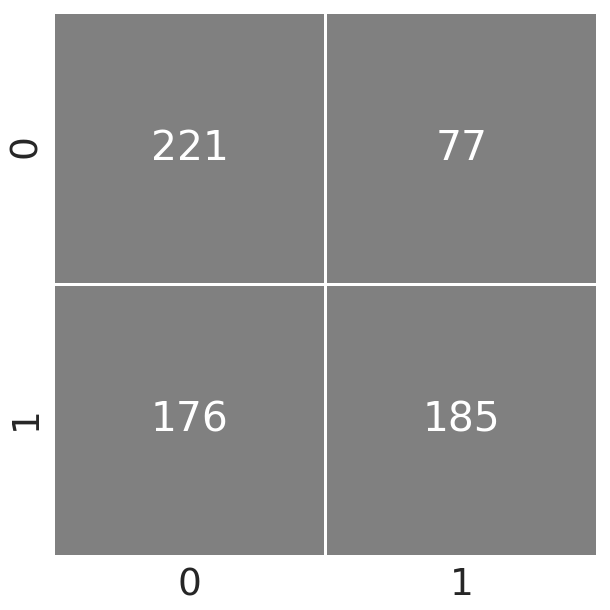The results seem similar. Below is the accuracy.

```round(accuracy_score(y_test, y_pred),2)
Out: 0.62```

About the same, our model generalizes even though it performs somewhat poorly.

Conclusion

This post provided an explanation of how to do a quadratic discriminant analysis using python. This is just another potential tool that may be useful for the data scientist.# Data Exploration Case Study: Credit Default

Exploratory data analysis is the main task of a Data Scientist with as much as 60% of their time being devoted to this task. As such, the majority of their time is spent on something that is rather boring compared to building models.This post will provide a simple example of how to analyze a dataset from the website called Kaggle. This dataset is looking at how is likely to default on their credit. The following steps will be conducted in this analysis.

1. Load the libraries and dataset
2. Deal with missing data
3. Some descriptive stats
4. Normality check
5. Model development

This is not an exhaustive analysis but rather a simple one for demonstration purposes. The dataset is available here

Here are some packages we will need

```import pandas as pd
import matplotlib.pyplot as plt
import seaborn as sns
from scipy.stats import norm
from sklearn import tree
from scipy import stats
from sklearn import metrics```

You can load the data with the code below

`df_train=pd.read_csv('/application_train.csv')`

You can examine what variables are available with the code below. This is not displayed here because it is rather long

```df_train.columns

Missing Data

I prefer to deal with missing data first because missing values can cause errors throughout the analysis if they are not dealt with immediately. The code below calculates the percentage of missing data in each column.

```total=df_train.isnull().sum().sort_values(ascending=False)
percent=(df_train.isnull().sum()/df_train.isnull().count()).sort_values(ascending=False)
missing_data=pd.concat([total,percent],axis=1,keys=['Total','Percent'])

Total   Percent
COMMONAREA_MEDI           214865  0.698723
COMMONAREA_AVG            214865  0.698723
COMMONAREA_MODE           214865  0.698723
NONLIVINGAPARTMENTS_MODE  213514  0.694330
NONLIVINGAPARTMENTS_MEDI  213514  0.694330```

Only the first five values are printed. You can see that some variables have a large amount of missing data. As such, they are probably worthless for inclusion in additional analysis. The code below removes all variables with any missing data.

```pct_null = df_train.isnull().sum() / len(df_train)
missing_features = pct_null[pct_null > 0.0].index
df_train.drop(missing_features, axis=1, inplace=True)```

You can use the .head() function if you want to see how  many variables are left.

Data Description & Visualization

For demonstration purposes, we will print descriptive stats and make visualizations of a few of the variables that are remaining.

```round(df_train['AMT_CREDIT'].describe())
Out:
count     307511.0
mean      599026.0
std       402491.0
min        45000.0
25%       270000.0
50%       513531.0
75%       808650.0
max      4050000.0

sns.distplot(df_train['AMT_CREDIT']```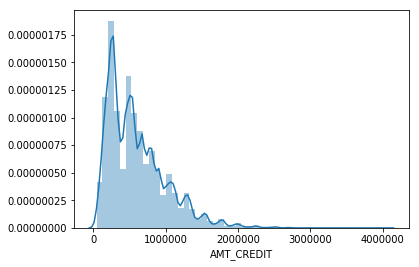```round(df_train['AMT_INCOME_TOTAL'].describe())
Out:
count       307511.0
mean        168798.0
std         237123.0
min          25650.0
25%         112500.0
50%         147150.0
75%         202500.0
max      117000000.0
sns.distplot(df_train['AMT_INCOME_TOTAL']```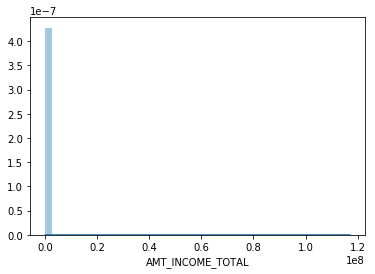I think you are getting the point. You can also look at categorical variables using the groupby() function.

We also need to address categorical variables in terms of creating dummy variables. This is so that we can develop a model in the future. Below is the code for dealing with all the categorical  variables and converting them to dummy variable’s

```df_train.groupby('NAME_CONTRACT_TYPE').count()
dummy=pd.get_dummies(df_train['NAME_CONTRACT_TYPE'])
df_train=pd.concat([df_train,dummy],axis=1)
df_train=df_train.drop(['NAME_CONTRACT_TYPE'],axis=1)

df_train.groupby('CODE_GENDER').count()
dummy=pd.get_dummies(df_train['CODE_GENDER'])
df_train=pd.concat([df_train,dummy],axis=1)
df_train=df_train.drop(['CODE_GENDER'],axis=1)

df_train.groupby('FLAG_OWN_CAR').count()
dummy=pd.get_dummies(df_train['FLAG_OWN_CAR'])
df_train=pd.concat([df_train,dummy],axis=1)
df_train=df_train.drop(['FLAG_OWN_CAR'],axis=1)

df_train.groupby('FLAG_OWN_REALTY').count()
dummy=pd.get_dummies(df_train['FLAG_OWN_REALTY'])
df_train=pd.concat([df_train,dummy],axis=1)
df_train=df_train.drop(['FLAG_OWN_REALTY'],axis=1)

df_train.groupby('NAME_INCOME_TYPE').count()
dummy=pd.get_dummies(df_train['NAME_INCOME_TYPE'])
df_train=pd.concat([df_train,dummy],axis=1)
df_train=df_train.drop(['NAME_INCOME_TYPE'],axis=1)

df_train.groupby('NAME_EDUCATION_TYPE').count()
dummy=pd.get_dummies(df_train['NAME_EDUCATION_TYPE'])
df_train=pd.concat([df_train,dummy],axis=1)
df_train=df_train.drop(['NAME_EDUCATION_TYPE'],axis=1)

df_train.groupby('NAME_FAMILY_STATUS').count()
dummy=pd.get_dummies(df_train['NAME_FAMILY_STATUS'])
df_train=pd.concat([df_train,dummy],axis=1)
df_train=df_train.drop(['NAME_FAMILY_STATUS'],axis=1)

df_train.groupby('NAME_HOUSING_TYPE').count()
dummy=pd.get_dummies(df_train['NAME_HOUSING_TYPE'])
df_train=pd.concat([df_train,dummy],axis=1)
df_train=df_train.drop(['NAME_HOUSING_TYPE'],axis=1)

df_train.groupby('ORGANIZATION_TYPE').count()
dummy=pd.get_dummies(df_train['ORGANIZATION_TYPE'])
df_train=pd.concat([df_train,dummy],axis=1)
df_train=df_train.drop(['ORGANIZATION_TYPE'],axis=1)```

You have to be careful with this because now you have many variables that are not necessary. For every categorical variable you must remove at least one category in order for the model to work properly.  Below we did this manually.

`df_train=df_train.drop(['Revolving loans','F','XNA','N','Y','SK_ID_CURR,''Student','Emergency','Lower secondary','Civil marriage','Municipal apartment'],axis=1)`

Below are some boxplots with the target variable and other variables in the dataset.

```f,ax=plt.subplots(figsize=(8,6))
fig=sns.boxplot(x=df_train['TARGET'],y=df_train['AMT_INCOME_TOTAL'])```There is a clear outlier there. Below is another boxplot with a different variable

```f,ax=plt.subplots(figsize=(8,6))
fig=sns.boxplot(x=df_train['TARGET'],y=df_train['CNT_CHILDREN'])```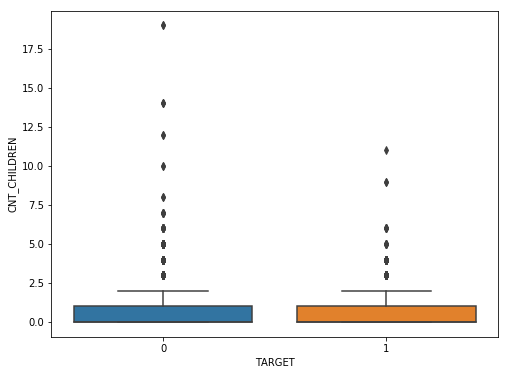It appears several people have more than 10 children. This is probably a typo.

Below is a correlation matrix using a heatmap technique

```corrmat=df_train.corr()
f,ax=plt.subplots(figsize=(12,9))
sns.heatmap(corrmat,vmax=.8,square=True)```The heatmap is nice but it is hard to really appreciate what is happening. The code below will sort the correlations from least to strongest, so we can remove high correlations.

```c = df_train.corr().abs()

s = c.unstack()
so = s.sort_values(kind="quicksort")

FLAG_DOCUMENT_12 FLAG_MOBIL 0.000005
FLAG_MOBIL FLAG_DOCUMENT_12 0.000005
Unknown FLAG_MOBIL 0.000005
FLAG_MOBIL Unknown 0.000005
Cash loans FLAG_DOCUMENT_14 0.000005```

The list is to long to show here but the following variables were removed for having a high correlation with other variables.

`df_train=df_train.drop(['WEEKDAY_APPR_PROCESS_START','FLAG_EMP_PHONE','REG_CITY_NOT_WORK_CITY','REGION_RATING_CLIENT','REG_REGION_NOT_WORK_REGION'],axis=1)`

Below we check a few variables for homoscedasticity, linearity, and normality  using plots and histograms

```sns.distplot(df_train['AMT_INCOME_TOTAL'],fit=norm)
fig=plt.figure()
res=stats.probplot(df_train['AMT_INCOME_TOTAL'],plot=plt)```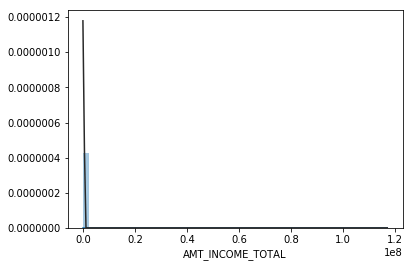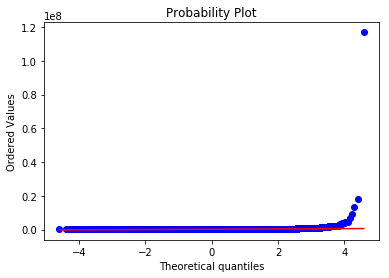This is not normal

```sns.distplot(df_train['AMT_CREDIT'],fit=norm)
fig=plt.figure()
res=stats.probplot(df_train['AMT_CREDIT'],plot=plt)```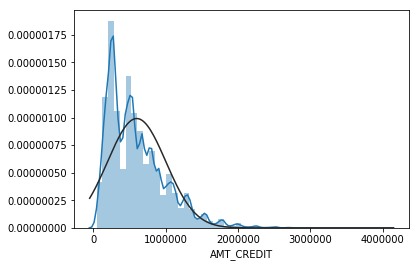This is not normal either. We could do transformations, or we can make a non-linear model instead.

Model Development

Now comes the easy part. We will make a decision tree using only some variables to predict the target. In the code below we make are X and y dataset.

```X=df_train[['Cash loans','DAYS_EMPLOYED','AMT_CREDIT','AMT_INCOME_TOTAL','CNT_CHILDREN','REGION_POPULATION_RELATIVE']]
y=df_train['TARGET']```

The code below fits are model and makes the predictions

```clf=tree.DecisionTreeClassifier(min_samples_split=20)
clf=clf.fit(X,y)
y_pred=clf.predict(X)```

Below is the confusion matrix followed by the accuracy

```print (pd.crosstab(y_pred,df_train['TARGET']))
TARGET       0      1
row_0
0       280873  18493
1         1813   6332
accuracy_score(y_pred,df_train['TARGET'])
Out: 0.933966589813047```

Lastly, we can look at the precision, recall, and f1 score

```print(metrics.classification_report(y_pred,df_train['TARGET']))
precision    recall  f1-score   support

0       0.99      0.94      0.97    299366
1       0.26      0.78      0.38      8145

micro avg       0.93      0.93      0.93    307511
macro avg       0.62      0.86      0.67    307511
weighted avg       0.97      0.93      0.95    307511```

This model looks rather good in terms of accuracy of the training set. It actually impressive that we could use so few variables from such a large dataset and achieve such a high degree of accuracy.

Conclusion

Data exploration and analysis is the primary task of a data scientist.  This post was just an example of how this can be approached. Of course, there are many other creative ways to do this but the simplistic nature of this analysis yielded strong results# RANSAC Regression in Python

RANSAC is an acronym for Random Sample Consensus. What this algorithm does is fit a regression model on a subset of data that the algorithm judges as inliers while removing outliers. This naturally improves the fit of the model due to the removal of some data points.The process that is used to determine inliers and outliers is described below.

1. The algorithm randomly selects a random amount of samples to be inliers in the model.
2. All data is used to fit the model and samples that fall with a certain tolerance are relabeled as inliers.
3. Model is refitted with the new inliers
4. Error of the fitted model vs the inliers is calculated
5. Terminate or go back to step 1 if a certain criterion of iterations or performance is not met.

In this post, we will use the tips data from the pydataset module. Our goal will be to predict the tip amount using two different models.

1. Model 1 will use simple regression and will include total bill as the independent variable and tips as the dependent variable
2. Model 2 will use multiple regression and  includes several independent variables and tips as the dependent variable

The process we will use to complete this example is as follows

1. Data preparation
2. Simple Regression Model fit
3. Simple regression visualization
4. Multiple regression model fit
5. Multiple regression visualization

Below are the packages we will need for this example

```import pandas as pd
from pydataset import data
from sklearn.linear_model import RANSACRegressor
from sklearn.linear_model import LinearRegression
import numpy as np
import matplotlib.pyplot as plt
from sklearn.metrics import mean_absolute_error
from sklearn.metrics import r2_score```

Data Preparation

For the data preparation, we need to do the following

2. Create X and y dataframes
3. Convert several categorical variables to dummy variables
4. Drop the original categorical variables from the X dataframe

Below is the code for these steps

```df=data('tips')
X,y=df[['total_bill','sex','size','smoker','time']],df['tip']
male=pd.get_dummies(X['sex'])
X['male']=male['Male']
smoker=pd.get_dummies(X['smoker'])
X['smoker']=smoker['Yes']
dinner=pd.get_dummies(X['time'])
X['dinner']=dinner['Dinner']
X=X.drop(['sex','time'],1)```

Most of this is self-explanatory, we first load the tips dataset and divide the independent and dependent variables into an X and y dataframe respectively. Next, we converted the sex, smoker, and dinner variables into dummy variables, and then we dropped the original categorical variables.

We can now move to fitting the first model that uses simple regression.

Simple Regression Model

For our model, we want to use total bill to predict tip amount. All this is done in the following steps.

1. Instantiate an instance of the RANSACRegressor. We the call LinearRegression function, and we also set the residual_threshold to 2 indicate how far an example has to be away from  2 units away from the line.
2. Next we fit the model
3. We predict the values
4. We calculate the r square  the mean absolute error

Below is the code for all of this.

```ransacReg1= RANSACRegressor(LinearRegression(),residual_threshold=2,random_state=0)
ransacReg1.fit(X[['total_bill']],y)
prediction1=ransacReg1.predict(X[['total_bill']])```
```r2_score(y,prediction1)
Out: 0.4381748268686979

mean_absolute_error(y,prediction1)
Out: 0.7552429811944833```

The r-square is 44% while the MAE is 0.75.  These values are most comparative and will be looked at again when we create the multiple regression model.

The next step is to make the visualization. The code below will create a plot that shows the X and y variables and the regression. It also identifies which samples are inliers and outliers. Te coding will not be explained because of the complexity of it.

```inlier=ransacReg1.inlier_mask_
outlier=np.logical_not(inlier)
line_X=np.arange(3,51,2)
line_y=ransacReg1.predict(line_X[:,np.newaxis])
plt.scatter(X[['total_bill']][inlier],y[inlier],c='lightblue',marker='o',label='Inliers')
plt.scatter(X[['total_bill']][outlier],y[outlier],c='green',marker='s',label='Outliers')
plt.plot(line_X,line_y,color='black')
plt.xlabel('Total Bill')
plt.ylabel('Tip')
plt.legend(loc='upper left')```Plot is self-explanatory as a handful of samples were considered outliers. We will now move to creating our multiple regression model.

Multiple Regression Model Development

The steps for making the model are mostly the same. The real difference takes place in make the plot which we will discuss in a moment. Below is the code for  developing the model.

```ransacReg2= RANSACRegressor(LinearRegression(),residual_threshold=2,random_state=0)
ransacReg2.fit(X,y)
prediction2=ransacReg2.predict(X)```
```r2_score(y,prediction2)
Out: 0.4298703800652126

mean_absolute_error(y,prediction2)
Out: 0.7649733201032204```

Things have actually gotten slightly worst in terms of r-square and MAE.

For the visualization, we cannot plot directly several variables t once. Therefore, we will compare the predicted values with the actual values. The better the correlated the better our prediction is. Below is the code for the visualization

```inlier=ransacReg2.inlier_mask_
outlier=np.logical_not(inlier)
line_X=np.arange(1,8,1)
line_y=(line_X[:,np.newaxis])
plt.scatter(prediction2[inlier],y[inlier],c='lightblue',marker='o',label='Inliers')
plt.scatter(prediction2[outlier],y[outlier],c='green',marker='s',label='Outliers')
plt.plot(line_X,line_y,color='black')
plt.xlabel('Predicted Tip')
plt.ylabel('Actual Tip')
plt.legend(loc='upper left')```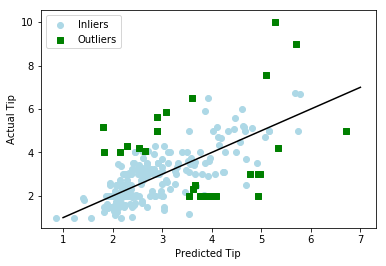The plots are mostly the same  as you cans see for yourself.

Conclusion

This post provided an example of how to use the RANSAC regressor algorithm. This algorithm will remove samples from the model based on a criterion you set. The biggest complaint about this algorithm is that it removes data from the model. Generally, we want to avoid losing data when developing models. In addition, the algorithm removes outliers objectively this is a problem because outlier removal is often subjective. Despite these flaws, RANSAC regression is another tool that can be use din machine learning.# Data Science Pipeline

One of the challenges of conducting a data analysis or any form of research is making decisions. You have to decide primarily two things

1. What to do
2. When to do it

People who are familiar with statistics may know what to do but may struggle with timing or when to do it. Others who are weaker when it comes to numbers may not knowwhat to do or when to do it. Generally, it is rare for someone to know when to do something but not know how to do it.

In this post, we will look at a process that that can be used to perform an analysis in the context of data science. Keep in mind that this is just an example and there are naturally many ways to perform an analysis. The purpose here is to provide some basic structure for people who are not sure of what to do and when. One caveat, this process is focused primarily on supervised learning which has a clearer beginning, middle, and end in terms of the process.

Generally, there are three steps that probably always take place when conducting a data analysis and they are as follows.

1. Data preparation (data mugging)
2. Model training
3. Model testing

Off course, it is much more complicated than this but this is the minimum. Within each of these steps there are several substeps, However, depending on the context, the substeps can be optional.

There is one pre-step that you have to consider. How you approach these three steps depends a great deal on the algorithm(s) you have in mind to use for developing different models. The assumptions and characteristics of one algorithm are different from another and shape how you prepare the data and develop models. With this in mind, we will go through each of these three steps.

Data Preparation

Data preparation involves several substeps. Some of these steps are necessary but general not all of them happen ever analysis. Below is a list of steps at this level

• Data mugging
• Scaling
• Normality
• Dimension reduction/feature extraction/feature selection
• Train, test, validation split

Data mugging is often the first step in data preparation and involves making sure your data is in a readable structure for your algorithm. This can involve changing the format of dates, removing punctuation/text, changing text into dummy variables or factors, combining tables, splitting tables, etc. This is probably the hardest and most unclear aspect of data science because the problems you will face will be highly unique to the dataset you are working with.

Scaling involves making sure all the variables/features are on the same scale. This is important because most algorithms are sensitive to the scale of the variables/features. Scaling can be done through normalization or standardization. Normalization reduces the variables to a range of 0 – 1. Standardization involves converting the examples in the variable to their respective z-score. Which one you use depends on the situation but normally it is expected to do this.

Normality is often an optional step because there are so many variables that can be involved with big data and data science in a given project. However, when fewer variables are involved checking for normality is doable with a few tests and some visualizations. If normality is violated various transformations can be used to deal with this problem. Keep mind that many machine learning algorithms are robust against the influence of non-normal data.

Dimension reduction involves reduce the number of variables that will be included in the final analysis. This is done through factor analysis or principal component analysis. This reduction  in the number of variables is also an example of feature extraction. In some context, feature extraction is the in goal in itself. Some algorithms make their own features such as neural networks through the use of hidden layer(s)

Feature selection is the process of determining which variables to keep for future analysis. This can be done through the use of regularization such or in smaller datasets with subset regression. Whether you extract or select features depends on the context.

After all this is accomplished, it is necessary to split the dataset. Traditionally, the data was split in two. This led to the development of a training set and a testing set. You trained the model on the training set and tested the performance on the test set.

However, now many analyst split the data into three parts to avoid overfitting the data to the test set. There is now a training a set, a validation set, and a testing set. The  validation set allows you to check the model performance several times. Once you are satisfied you use the test set once at the end.

Once the data is prepared, which again is perhaps the most difficult part, it is time to train the model.

Model training

Model training involves several substeps as well

1. Determine the metric(s) for success
2. Creating a grid of several hyperparameter values
3. Cross-validation
4. Selection of the most appropriate hyperparameter values

The first thing you have to do and this is probably required is determined how you will know if your model is performing well. This involves selecting a metric. It can be accuracy for classification or mean squared error for a regression model or something else. What you pick depends on your goals. You use these metrics to determine the best algorithm and hyperparameters settings.

Most algorithms have some sort of hyperparameter(s). A hyperparameter is a value or estimate that the algorithm cannot learn and must be set by you. Since there is no way of knowing what values to select it is common practice to have several values tested and see which one is the best.

Cross-validation is another consideration. Using cross-validation always you to stabilize the results through averaging the results of the model over several folds of the data if you are using k-folds cross-validation. This also helps to improve the results of the hyperparameters as well.  There are several types of cross-validation but k-folds is probably best initially.

The information for the metric, hyperparameters, and cross-validation are usually put into  a grid that then runs the model. Whether you are using R or Python the printout will tell you which combination of hyperparameters is the best based on the metric you determined.

Validation test

When you know what your hyperparameters are you can now move your model to validation or straight to testing. If you are using a validation set you asses your models performance by using this new data. If the results are satisfying based on your metric you can move to testing. If not, you may move back and forth between training and the validation set making the necessary adjustments.

Test set

The final step is testing the model. You want to use the testing dataset as little as possible. The purpose here is to see how your model generalizes to data it has not seen before. There is little turning back after this point as there is an intense danger of overfitting now. Therefore, make sure you are ready before playing with the test data.

Conclusion

This is just one approach to conducting data analysis. Keep in mind the need to prepare data, train your model, and test it. This is the big picture for a somewhat complex process# Gradient Boosting Classification in Python

Gradient Boosting is an alternative form of boosting to AdaBoost. Many consider gradient boosting to be a better performer than adaboost. Some differences between the two algorithms is that gradient boosting uses optimization for weight the estimators. Like adaboost, gradient boosting can be used for most algorithms but is commonly associated with decision trees.

In addition, gradient boosting requires several additional hyperparameters such as max depth and subsample. Max depth has to do with the number of nodes in a tree. The higher the number the purer the classification become. The downside to this is the risk of overfitting.

Subsampling has to do with the proportion of the sample that is used for each estimator. This can range from a decimal value up until the whole number 1. If the value is set to 1 it becomes stochastic gradient boosting.

This post is focused on classification. To do this, we will use the cancer dataset from the pydataset library. Our goal will be to predict the status of patients (alive or dead) using the available independent variables. The steps we will use are as follows.

1. Data preparation
2. Baseline decision tree model
3. Hyperparameter tuning

Below is some initial code.

`from sklearn.ensemble import GradientBoostingClassifier from sklearn import tree from sklearn.model_selection import GridSearchCV import numpy as np from pydataset import data import pandas as pd from sklearn.model_selection import cross_val_score from sklearn.model_selection import KFold`

Data Preparation

The data preparation is simple in this situtation. All we need to do is load are dataset, dropping missing values, and create our X dataset and y dataset. All this happens in the code below.

`df=data('cancer').dropna() X=df[['time','sex','ph.karno','pat.karno','meal.cal','wt.loss']] y=df['status'] `

We will now develop our baseline decision tree model.

Baseline Model

The purpose of the baseline model is to have something to compare our gradient boosting model to. The strength of a model is always relative to some other model, so we need to make at least two, so we can say one is better than the other.

The criteria for better in this situation is accuracy. Therefore, we will make a decision tree model, but we will manipulate the max depth of the tree to create 9 different baseline models. The best accuracy model will be the baseline model.

To achieve this, we need to use a for loop to make python make several decision trees. We also need to set the parameters for the cross validation by calling KFold(). Once this is done, we print the results for the 9 trees. Below is the code and results.

`crossvalidation=KFold(n_splits=10,shuffle=True,random_state=1)for depth in range (1,10):     tree_classifier=tree.DecisionTreeClassifier(max_depth=depth,random_state=1)     if tree_classifier.fit(X,y).tree_.max_depth<depth:         break     score=np.mean(cross_val_score(tree_classifier,X,y,scoring='accuracy', cv=crossvalidation,n_jobs=1))     print(depth, score) 1 0.71875 2 0.6477941176470589 3 0.6768382352941177 4 0.6698529411764707 5 0.6584558823529412 6 0.6525735294117647 7 0.6283088235294118 8 0.6573529411764706 9 0.6577205882352941`

It appears that when the max depth is limited to 1 that we get the best accuracy at almost 72%. This will be our baseline for comparison. We will now tune the parameters for the gradient boosting algorithm

Hyperparameter Tuning

There are several hyperparameters we need to tune. The ones we will tune are as follows

• number of estimators
• learning rate
• subsample
• max depth

First, we will create an instance of the gradient boosting classifier. Second, we will create our grid for the search. It is inside this grid that we set several values for each hyperparameter. Then we call GridSearchCV and place the instance of the gradient boosting classifier, the grid, the cross validation values from mad earlier, and n_jobs all together in one place. Below is the code for this.

`GBC=GradientBoostingClassifier() search_grid={'n_estimators':[500,1000,2000],'learning_rate':[.001,0.01,.1],'max_depth':[1,3,5],'subsample':[.5,.75,1],'random_state':}search=GridSearchCV(estimator=GBC,param_grid=search_grid,scoring='accuracy',n_jobs=1,cv=crossvalidation)`

You can now run your model by calling .fit(). Keep in mind that there are several hyperparameters. This means that it might take some time to run the calculations. It is common to find values for max depth, subsample, and number of estimators first. Then as second run through is done to find the learning rate. In our example, we are doing everything at once which is why it takes longer. Below is the code with the out for best parameters and best score.

`search.fit(X,y)search.best_params_ Out:  {'learning_rate': 0.01,  'max_depth': 5,  'n_estimators': 2000,  'random_state': 1,  'subsample': 0.75} search.best_score_ Out: 0.7425149700598802`

You can see what the best hyperparameters are for yourself. In addition, we see that when these parameters were set we got an accuracy of 74%. This is superior to our baseline model. We will now see if we can replicate these numbers when we use them for our Gradient Boosting model.

Below is the code and results for the model with the predetermined hyperparameter values.

`ada2=GradientBoostingClassifier(n_estimators=2000,learning_rate=0.01,subsample=.75,max_depth=5,random_state=1) score=np.mean(cross_val_score(ada2,X,y,scoring='accuracy',cv=crossvalidation,n_jobs=1)) score Out: 0.742279411764706`

You can see that the results are similar. This is just additional information that the gradient boosting model does outperform the baseline decision tree model.

Conclusion

This post provided an example of what gradient boosting classification can do for a model. With its distinct characteristics gradient boosting is generally a better performing boosting algorithm in comparison to AdaBoost.This post will share how to use the adaBoost algorithm for regression in Python. What boosting does is that it makes multiple models in a sequential manner. Each newer model tries to successful predict what older models struggled with. For regression, the average of the models are used for the predictions.  It is often most common to use boosting with decision trees but this approach can be used with any machine learning algorithm that deals with supervised learning.

Boosting is associated with ensemble learning because several models are created that are averaged together. An assumption of boosting, is that combining several weak models can make one really strong and accurate model.

For our purposes, we will be using adaboost classification to improve the performance of a decision tree in python. We will use the cancer dataset from the pydataset library. Our goal will be to predict the weight loss of a patient based on several independent variables. The steps of this process are as follows.

1. Data preparation
2. Regression decision tree baseline model
3. Hyperparameter tuning of Adaboost regression model

Below is some initial code

`from sklearn.ensemble import AdaBoostRegressor from sklearn import tree from sklearn.model_selection import GridSearchCV import numpy as np from pydataset import data import pandas as pd from sklearn.model_selection import cross_val_score from sklearn.model_selection import train_test_split from sklearn.model_selection import KFold from sklearn.metrics import mean_squared_error`

Data Preparation

There is little data preparation for this example. All we need to do is load the data and create the X and y datasets. Below is the code.

`df=data('cancer').dropna() X=df[['time','sex','ph.karno','pat.karno','status','meal.cal']] y=df['wt.loss']`

We will now proceed to creating the baseline regression decision tree model.

Baseline Regression Tree Model

The purpose of the baseline model is for comparing it to the performance of our model that utilizes adaBoost. In order to make this model we need to Initiate a Kfold cross-validation. This will help in stabilizing the results. Next we will create a for loop so that we can create several trees that vary based on their depth. By depth, it is meant how far the tree can go to purify the classification. More depth often leads to a higher likelihood of overfitting.

Finally, we will then print the results for each tree. The criteria used for judgment is the mean squared error. Below is the code and results

`for depth in range (1,10):     tree_regressor=tree.DecisionTreeRegressor(max_depth=depth,random_state=1)     if tree_regressor.fit(X,y).tree_.max_depth<depth:         break     score=np.mean(cross_val_score(tree_regressor,X,y,scoring='neg_mean_squared_error', cv=crossvalidation,n_jobs=1))     print(depth, score) 1 -193.55304528235052 2 -176.27520747356175 3 -209.2846723461564 4 -218.80238479654003 5 -222.4393459885871 6 -249.95330609042858 7 -286.76842138165705 8 -294.0290706405905 9 -287.39016236497804`

Looks like a tree with a depth of 2 had the lowest amount of error. We can now move to tuning the hyperparameters for the adaBoost algorithm.

Hyperparameter Tuning

For hyperparameter tuning we need to start by initiating our AdaBoostRegresor() class. Then we need to create our grid. The grid will address two hyperparameters which are the number of estimators and the learning rate. The number of estimators tells Python how many models to make and the learning indicates how each tree contributes to the overall results. There is one more parameters which is random_state but this is just for setting the seed and never changes.

After making the grid, we need to use the GridSearchCV function to finish this process. Inside this function you have to set the estimator which is adaBoostRegressor, the parameter grid which we just made, the cross validation which we made when we created the baseline model, and the n_jobs which allocates resources for the calculation. Below is the code.

`ada=AdaBoostRegressor()search_grid={'n_estimators':[500,1000,2000],'learning_rate':[.001,0.01,.1],'random_state':}search=GridSearchCV(estimator=ada,param_grid=search_grid,scoring='neg_mean_squared_error',n_jobs=1,cv=crossvalidation) `

Next, we can run the model with the desired grid in place. Below is the code for fitting the mode as well as the best parameters and the score to expect when using the best parameters.

`search.fit(X,y)search.best_params_ Out: {'learning_rate': 0.01, 'n_estimators': 500, 'random_state': 1} search.best_score_ Out: -164.93176650920856`

The best mix of hyperparameters is a learning rate of 0.01 and 500 estimators. This mix led to a mean error score of 164, which is a little lower than our single decision tree of 176. We will see how this works when we run our model with the refined hyperparameters.

Below is our model but this time with the refined hyperparameters.

`ada2=AdaBoostRegressor(n_estimators=500,learning_rate=0.001,random_state=1)score=np.mean(cross_val_score(ada2,X,y,scoring='neg_mean_squared_error',cv=crossvalidation,n_jobs=1)) score Out: -174.52604137201791`

You can see the score is not as good but it is within reason.

Conclusion

In this post, we explored how to use the AdaBoost algorithm for regression. Employing this algorithm can help to strengthen a model in many ways at times.# Web Scraping with R

In this post we are going to learn how to do web scrapping with R.Web scraping is a process for extracting data from a website. We have all done web scraping before. For example, whenever you copy and paste something from a website into another document such as Word this is an example of web scraping. Technically, this is an example of manual web scraping. The main problem with manual web scraping is that it is labor intensive and takes a great deal of time.Another problem with web scraping is that the data can come in an unstructured manner. This means that you have to organize it in some way in order to conduct a meaningful analysis. This also means that you must have a clear purpose for what you are scraping along with answerable questions. Otherwise, it is easy to become confused quickly when web scraping

Therefore, we will learn how to automate this process using R. We will need the help of the “rest” and “xml2” packages to do this. Below is some initial code

``library(rvest);library(xml2)``

For our example, we are going to scrape the titles and prices of books from a webpage on Amazon. When simply want to make an organized data frame. The first thing we need to do is load the URL into R and have R read the website using the “read_html” function. The code is below.

``````url<-'https://www.amazon.com/s/ref=nb_sb_noss?url=search-alias%3Daps&field-keywords=books'

We now need to specifically harvest the titles from the webpage as this is one of our goals. There are at least two ways to do this. If you are an expert in HTML you can find the information by inspecting the page’s HTML. Another way is to the selectorGadget extension available in Chrome. When using this extension you simply click on the information you want to inspect and it gives you the CSS selector for that particular element. This is shown belowThe green highlight is the CSS selector that you clicked on. The yellow represents all other elements that have the same CSS selector. The red represents what you do not want to be included. In this picture, I do not want the price because I want to scrape this separately.

Once you find your information you want to copy the CSS element information in the bar at the bottom of the picture. This information is then pasted into R and use the “html_nodes” function to pull this specific information from the webpage.

``bookTitle<- html_nodes(webpage,'.a-link-normal .a-size-base')``

We now need to convert this information to text and we are done.

``title <- html_text(bookTitle, trim = TRUE) ``

Next, we repeat this process for the price.

``````bookPrice<- html_nodes(webpage,'.acs_product-price__buying')
price <- html_text(bookPrice, trim = TRUE) ``````

Lastly, we make our data frame with all of our information.

``````books<-as.data.frame(title)
books\$price<-price``````

With this done we can do basic statistical analysis such as the mean, standard deviation, histogram, etc. This was not a complex example but the basics of pulling data was provided. Below is what the first few entries of the data frame look like.

``````head(books)
##                                   title  price
## 1                          Silent Child \$17.95
## 2 Say You're Sorry (Morgan Dane Book 1)  \$4.99
## 3                     A Wrinkle in Time \$19.95
## 4                       The Whiskey Sea  \$3.99
## 5            Speaking in Bones: A Novel  \$2.99
## 6 Harry Potter and the Sorcerer's Stone  \$8.99``````

Conclusion

Web scraping using automated tools saves time and increases the possibilities of data analysis. The most important thing to remember is to understand what exactly it is you want to know. Otherwise, you will quickly become lost due to the overwhelming amounts of available information.

# aggregate Function in R VIDEO

Using the aggregate function in R.

# Creating Subgroups of Data in R VIDEO

Create subgroups in R

# Subsetting Data in R VIDEO

Subsetting data in R

# Getting Data Out of R Video

Getting data out of R

# Importing Data into R VIDEO

Importing data into R

for loops in R

# APA Tables in R

Anybody who has ever had to do any writing for academic purposes or in industry has had to deal with APA formatting. The rules and expectations seem to be endless and always changing. If you are able to maneuver the endless list of rules you still have to determine what to report and how when writing an article.

There is a package in R that can at least take away the mystery of how to report ANOVA, correlation, and regression tables. This package is called “apaTables”. In this post, we will look at how to use this package for making tables that are formatted according to APA.

We are going to create examples of ANOVA, correlation, and regression tables using the ‘mtcars’ dataset. Below is the initial code that we need to begin.

``````library(apaTables)
data("mtcars")``````

ANOVA

We will begin with the results of ANOVA. In order for this to be successful, you have to use the “lm” function to create the model. If you are familiar with ANOVA and regression this should not be surprising as they both find the same answer using different approaches. After the “lm” function you must use the “filename” argument and give the output a name in quotations. This file will be saved in your R working directory. You can also provide other information such as the table number and confidence level if you desire.

There will be two outputs in our code. The output to the console is in R. A second output will be in a word doc. Below is the code.

``apa.aov.table(lm(mpg~cyl,mtcars),filename = "Example1.doc",table.number = 1)``
``````##
##
## Table 1
##
## ANOVA results using mpg as the dependent variable
##
##
##    Predictor      SS df      MS      F    p partial_eta2
##  (Intercept) 3429.84  1 3429.84 333.71 .000
##          cyl  817.71  1  817.71  79.56 .000          .73
##        Error  308.33 30   10.28
##  CI_90_partial_eta2
##
##          [.56, .80]
##
##
## Note: Values in square brackets indicate the bounds of the 90% confidence interval for partial eta-squared``````

Here is the word doc outputPerhaps you are beginning to see the beauty of using this package and its functions. The “apa.aov.table”” function provides a nice table that requires no formatting by the researcher.

You can even make a table of the means and standard deviations of ANOVA. This is similar to what you would get if you used the “aggregate” function. Below is the code.

``apa.1way.table(cyl, mpg,mtcars,filename = "Example2.doc",table.number = 2)``
``````##
##
## Table 2
##
## Descriptive statistics for mpg as a function of cyl.
##
##  cyl     M   SD
##    4 26.66 4.51
##    6 19.74 1.45
##    8 15.10 2.56
##
## Note. M and SD represent mean and standard deviation, respectively.
## ``````

Here is what it looks like in wordCorrelation

We will now look at an example of a correlation table. The function for this is “apa.cor.table”. This function works best with only a few variables. Otherwise, the table becomes bigger than a single sheet of paper. In addition, you probably will want to suppress the confidence intervals to save space. There are other arguments that you can explore on your own. Below is the code

``apa.cor.table(mtcars,filename = "Example3.doc",table.number = 3,show.conf.interval = F)``
``````##
##
## Table 3
##
## Means, standard deviations, and correlations
##
##
##   Variable M      SD     1      2      3      4      5      6      7
##   1. mpg   20.09  6.03
##
##   2. cyl   6.19   1.79   -.85**
##
##   3. disp  230.72 123.94 -.85** .90**
##
##   4. hp    146.69 68.56  -.78** .83**  .79**
##
##   5. drat  3.60   0.53   .68**  -.70** -.71** -.45**
##
##   6. wt    3.22   0.98   -.87** .78**  .89**  .66**  -.71**
##
##   7. qsec  17.85  1.79   .42*   -.59** -.43*  -.71** .09    -.17
##
##   8. vs    0.44   0.50   .66**  -.81** -.71** -.72** .44*   -.55** .74**
##
##   9. am    0.41   0.50   .60**  -.52** -.59** -.24   .71**  -.69** -.23
##
##   10. gear 3.69   0.74   .48**  -.49** -.56** -.13   .70**  -.58** -.21
##
##   11. carb 2.81   1.62   -.55** .53**  .39*   .75**  -.09   .43*   -.66**
##
##   8      9     10
##
##
##
##
##
##
##
##
##
##
##
##
##
##
##
##
##   .17
##
##   .21    .79**
##
##   -.57** .06   .27
##
##
## Note. * indicates p < .05; ** indicates p < .01.
## M and SD are used to represent mean and standard deviation, respectively.
## ``````

Here is the word doc results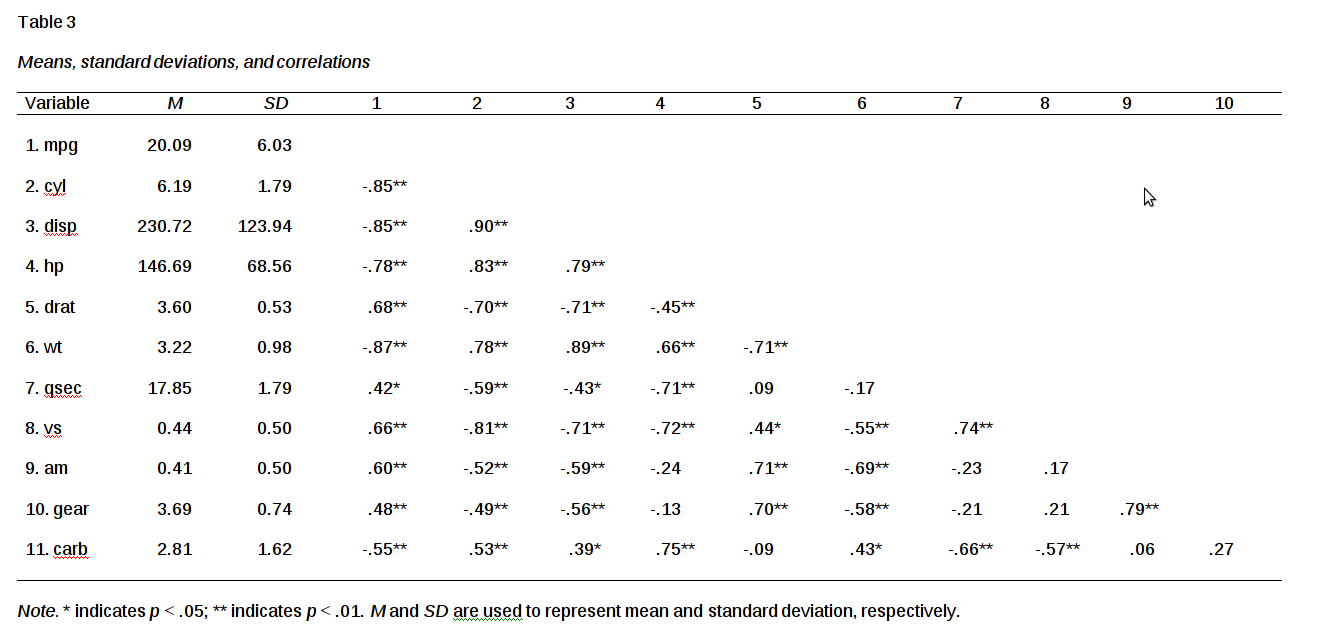If you run this code at home and open the word doc in Word you will not see variables 9 and 10 because the table is too big by itself for a single page. I hade to resize it manually. One way to get around this is to delate the M and SD column and place those as rows below the table.

Regression

Our final example will be a regression table. The code is as follows

``apa.reg.table(lm(mpg~disp,mtcars),filename = "Example4",table.number = 4)``
``````##
##
## Table 4
##
## Regression results using mpg as the criterion
##
##
##    Predictor       b       b_95%_CI  beta    beta_95%_CI sr2 sr2_95%_CI
##  (Intercept) 29.60** [27.09, 32.11]
##         disp -0.04** [-0.05, -0.03] -0.85 [-1.05, -0.65] .72 [.51, .81]
##
##
##
##       r             Fit
##
##  -.85**
##             R2 = .718**
##         95% CI[.51,.81]
##
##
## Note. * indicates p < .05; ** indicates p < .01.
## A significant b-weight indicates the beta-weight and semi-partial correlation are also significant.
## b represents unstandardized regression weights; beta indicates the standardized regression weights;
## sr2 represents the semi-partial correlation squared; r represents the zero-order correlation.
## Square brackets are used to enclose the lower and upper limits of a confidence interval.
## ``````

Here are the results in wordYou can also make regression tables that have multiple blocks or models. Below is an example

``apa.reg.table(lm(mpg~disp,mtcars),lm(mpg~disp+hp,mtcars),filename = "Example5",table.number = 5)``
``````##
##
## Table 5
##
## Regression results using mpg as the criterion
##
##
##    Predictor       b       b_95%_CI  beta    beta_95%_CI sr2  sr2_95%_CI
##  (Intercept) 29.60** [27.09, 32.11]
##         disp -0.04** [-0.05, -0.03] -0.85 [-1.05, -0.65] .72  [.51, .81]
##
##
##
##  (Intercept) 30.74** [28.01, 33.46]
##         disp -0.03** [-0.05, -0.02] -0.62 [-0.94, -0.31] .15  [.00, .29]
##           hp   -0.02  [-0.05, 0.00] -0.28  [-0.59, 0.03] .03 [-.03, .09]
##
##
##
##       r             Fit        Difference
##
##  -.85**
##             R2 = .718**
##         95% CI[.51,.81]
##
##
##  -.85**
##  -.78**
##             R2 = .748**    Delta R2 = .03
##         95% CI[.54,.83] 95% CI[-.03, .09]
##
##
## Note. * indicates p < .05; ** indicates p < .01.
## A significant b-weight indicates the beta-weight and semi-partial correlation are also significant.
## b represents unstandardized regression weights; beta indicates the standardized regression weights;
## sr2 represents the semi-partial correlation squared; r represents the zero-order correlation.
## Square brackets are used to enclose the lower and upper limits of a confidence interval.
## ``````

Here is the word doc versionConculsion

This is a real time saver for those of us who need to write and share statistical information.

# Vectorization of function in R VIDEO

Vectorization of function in R

# If Else Statements in Functions R VIDEO

If else statements in functions in R

# If Statements with Functions in R VIDEO

If Statements in R

# Arguments and Functions in R VIDEO

Arguments and functions in R

# Intro to Functions in R VIDEOS

R functions intro

# Intro to List VIDEO

Introduction to using List

# Manipulating Dataframes in R VIDEO

Manipulating Dataframes in R

# Intro to Dataframes in R VIDEO

Intro to dataframes in R

# Local Regression in R

Local regression uses something similar to nearest neighbor classification to generate a regression line. In local regression, nearbyobservations are used to fit the line rather than all observations. It is necessary to indicate the percentage of the observations you want R to use for fitting the local line. The name for this hyperparameter is the span. The higher the span the smoother the line becomes.

Local regression is great one there are only a handful of independent variables in the model. When the total number of variables becomes too numerous the model will struggle. As such, we will only fit a bivariate model. This will allow us to process the model and to visualize it.

In this post, we will use the “Clothing” dataset from the “Ecdat” package and we will examine innovation (inv2) relationship with total sales (tsales). Below is some initial code.

``library(Ecdat)``
``````data(Clothing)
str(Clothing)``````
``````## 'data.frame':    400 obs. of  13 variables:
##  \$ tsales : int  750000 1926395 1250000 694227 750000 400000 1300000 495340 1200000 495340 ...
##  \$ sales  : num  4412 4281 4167 2670 15000 ...
##  \$ margin : num  41 39 40 40 44 41 39 28 41 37 ...
##  \$ nown   : num  1 2 1 1 2 ...
##  \$ nfull  : num  1 2 2 1 1.96 ...
##  \$ npart  : num  1 3 2.22 1.28 1.28 ...
##  \$ naux   : num  1.54 1.54 1.41 1.37 1.37 ...
##  \$ hoursw : int  76 192 114 100 104 72 161 80 158 87 ...
##  \$ hourspw: num  16.8 22.5 17.2 21.5 15.7 ...
##  \$ inv1   : num  17167 17167 292857 22207 22207 ...
##  \$ inv2   : num  27177 27177 71571 15000 10000 ...
##  \$ ssize  : int  170 450 300 260 50 90 400 100 450 75 ...
##  \$ start  : num  41 39 40 40 44 41 39 28 41 37 ...``````

There is no data preparation in this example. The first thing we will do is fit two different models that have different values for the span hyperparameter. “fit” will have a span of .41 which means it will use 41% of the nearest examples. “fit2” will use .82. Below is the code.

``````fit<-loess(tsales~inv2,span = .41,data = Clothing)
fit2<-loess(tsales~inv2,span = .82,data = Clothing)``````

In the code above, we used the “loess” function to fit the model. The “span” argument was set to .41 and .82.

We now need to prepare for the visualization. We begin by using the “range” function to find the distance from the lowest to the highest value. Then use the “seq” function to create a grid. Below is the code.

``````inv2lims<-range(Clothing\$inv2)
inv2.grid<-seq(from=inv2lims,to=inv2lims)``````

The information in the code above is for setting our x-axis in the plot. We are now ready to fit our model. We will fit the models and draw each regression line.

``````plot(Clothing\$inv2,Clothing\$tsales,xlim=inv2lims)
lines(inv2.grid,predict(fit,data.frame(inv2=inv2.grid)),col='blue',lwd=3)
lines(inv2.grid,predict(fit2,data.frame(inv2=inv2.grid)),col='red',lwd=3)``````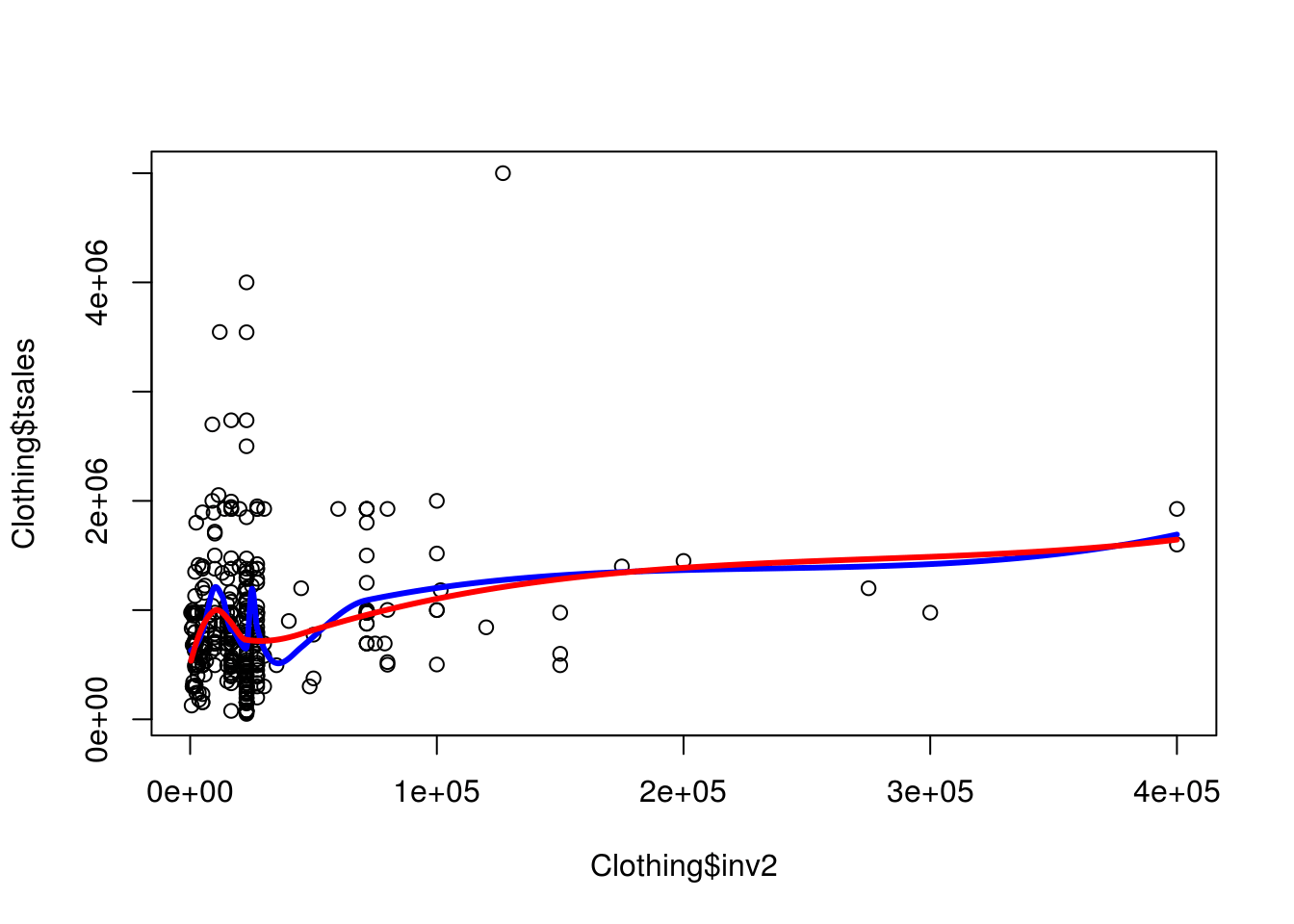Not much difference in the two models. For our final task, we will predict with our “fit” model using all possible values of “inv2” and also fit the confidence interval lines.

``````pred<-predict(fit,newdata=inv2.grid,se=T)
plot(Clothing\$inv2,Clothing\$tsales)
lines(inv2.grid,pred\$fit,col='red',lwd=3)
lines(inv2.grid,pred\$fit+2*pred\$se.fit,lty="dashed",lwd=2,col='blue')
lines(inv2.grid,pred\$fit-2*pred\$se.fit,lty="dashed",lwd=2,col='blue')``````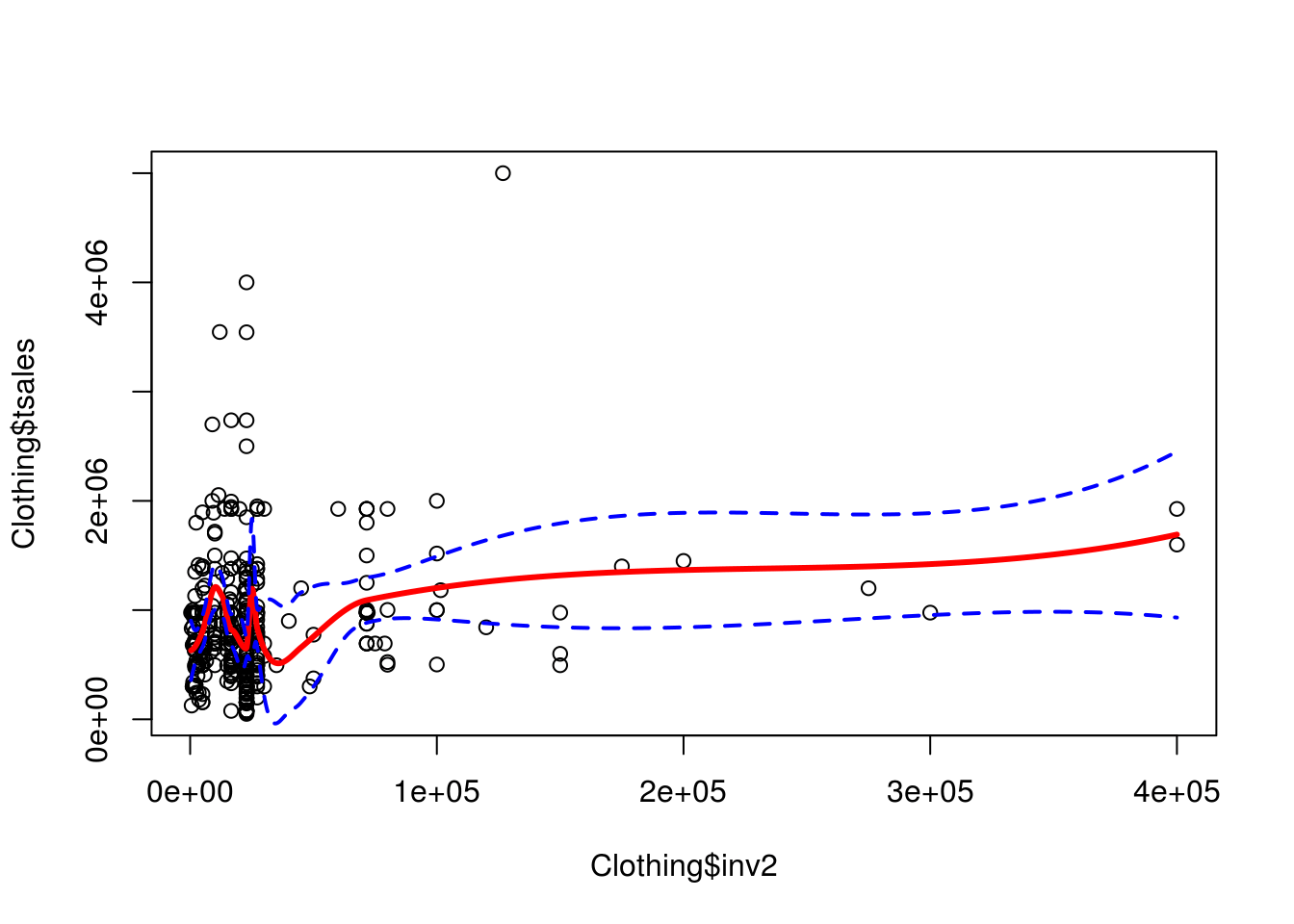Conclusion

Local regression provides another way to model complex non-linear relationships in low dimensions. The example here provides just the basics of how this is done is much more complicated than described here.

# Smoothing Splines in R

This post will provide information on smoothing splines. Smoothing splines are used in regression when we want to reduce the residual sum of squares by adding more flexibility to the regression line without allowing too much overfitting.In order to do this, we must tune the parameter called the smoothing spline. The smoothing spline is essentially a natural cubic spline with a knot at every unique value of x in the model. Having this many knots can lead to severe overfitting. This is corrected for by controlling the degrees of freedom through the parameter called lambda. You can manually set this value or select it through cross-validation.

We will now look at an example of the use of smoothing splines with the “Clothing” dataset from the “Ecdat” package. We want to predict “tsales” based on the use of innovation in the stores. Below is some initial code.

``library(Ecdat)``
``````data(Clothing)
str(Clothing)``````
``````## 'data.frame':    400 obs. of  13 variables:
##  \$ tsales : int  750000 1926395 1250000 694227 750000 400000 1300000 495340 1200000 495340 ...
##  \$ sales  : num  4412 4281 4167 2670 15000 ...
##  \$ margin : num  41 39 40 40 44 41 39 28 41 37 ...
##  \$ nown   : num  1 2 1 1 2 ...
##  \$ nfull  : num  1 2 2 1 1.96 ...
##  \$ npart  : num  1 3 2.22 1.28 1.28 ...
##  \$ naux   : num  1.54 1.54 1.41 1.37 1.37 ...
##  \$ hoursw : int  76 192 114 100 104 72 161 80 158 87 ...
##  \$ hourspw: num  16.8 22.5 17.2 21.5 15.7 ...
##  \$ inv1   : num  17167 17167 292857 22207 22207 ...
##  \$ inv2   : num  27177 27177 71571 15000 10000 ...
##  \$ ssize  : int  170 450 300 260 50 90 400 100 450 75 ...
##  \$ start  : num  41 39 40 40 44 41 39 28 41 37 ...``````

We are going to create three models. Model one will have 70 degrees of freedom, model two will have 7, and model three will have the number of degrees of freedom are determined through cross-validation. Below is the code.

``````fit1<-smooth.spline(Clothing\$inv2,Clothing\$tsales,df=57)
fit2<-smooth.spline(Clothing\$inv2,Clothing\$tsales,df=7)
fit3<-smooth.spline(Clothing\$inv2,Clothing\$tsales,cv=T)``````
``````## Warning in smooth.spline(Clothing\$inv2, Clothing\$tsales, cv = T): cross-
## validation with non-unique 'x' values seems doubtful``````
``(data.frame(fit1\$df,fit2\$df,fit3\$df))``
``````##   fit1.df  fit2.df  fit3.df
## 1      57 7.000957 2.791762``````

In the code above we used the “smooth.spline” function which comes with base r.Notice that we did not use the same coding syntax as the “lm” function calls for. The code above also indicates the degrees of freedom for each model.  You can see that for “fit3” the cross-validation determine that 2.79 was the most appropriate degrees of freedom. In addition, if you type in the following code.

``sapply(data.frame(fit1\$x,fit2\$x,fit3\$x),length)``
``````## fit1.x fit2.x fit3.x
##     73     73     73``````

You will see that there are only 73 data points in each model. The “Clothing” dataset has 400 examples in it. The reason for this reduction is that the “smooth.spline” function only takes unique values from the original dataset. As such, though there are 400 examples in the dataset only 73 of them are unique.

Next, we plot our data and add regression lines

``````plot(Clothing\$inv2,Clothing\$tsales)
lines(fit1,col='red',lwd=3)
lines(fit2,col='green',lwd=3)
lines(fit3,col='blue',lwd=3)
legend('topright',lty=1,col=c('red','green','blue'),c("df = 57",'df=7','df=CV 2.8'))``````You can see that as the degrees of freedom increase so does the flexibility in the line. The advantage of smoothing splines is to have a more flexible way to assess the characteristics of a dataset.

# Polynomial Spline Regression in R

Normally, when least squares regression is used, you fit one line to the model. However, sometimes you may want enough flexibility that you fit different lines over different regions of your independent variable. This process of fitting different lines over different regions of X is known as Regression Splines.How this works is that there are different coefficient values based on the regions of X. As the researcher, you can set the cutoff points for each region. The cutoff point is called a “knot.” The more knots you use the more flexible the model becomes because there are fewer data points with each range allowing for more variability.

We will now go through an example of polynomial regression splines. Remeber that polynomial means that we will have a curved line as we are using higher order polynomials. Our goal will be to predict total sales based on the amount of innovation a store employs. We will use the “Ecdat” package and the “Clothing” dataset. In addition, we will need the “splines” package. The code is as follows.

``library(splines);library(Ecdat)``
``data(Clothing)``

We will now fit our model. We must indicate the number and placement of the knots. This is commonly down at the 25th 50th and 75th percentile. Below is the code

``fit<-lm(tsales~bs(inv2,knots = c(12000,60000,150000)),data = Clothing)``

In the code above we used the traditional “lm” function to set the model. However, we also used the “bs” function which allows us to create our spline regression model. The argument “knots” was set to have three different values. Lastly, the dataset was indicated.

Remember that the default spline model in R is a third-degree polynomial. This is because it is hard for the eye to detect the discontinuity at the knots.

We now need X values that we can use for prediction purposes. In the code below we first find the range of the “inv2” variable. We then create a grid that includes all the possible values of “inv2” in increments of 1. Lastly, we use the “predict” function to develop the prediction model. We set the “se” argument to true as we will need this information. The code is below.

``````inv2lims<-range(Clothing\$inv2)
inv2.grid<-seq(from=inv2lims,to=inv2lims)
pred<-predict(fit,newdata=list(inv2=inv2.grid),se=T)``````

We are now ready to plot our model. The code below graphs the model and includes the regression line (red), confidence interval (green), as well as the location of each knot (blue)

``````plot(Clothing\$inv2,Clothing\$tsales,main="Regression Spline Plot")
lines(inv2.grid,pred\$fit,col='red',lwd=3)
lines(inv2.grid,pred\$fit+2*pred\$se.fit,lty="dashed",lwd=2,col='green')
lines(inv2.grid,pred\$fit-2*pred\$se.fit,lty="dashed",lwd=2,col='green')
segments(12000,0,x1=12000,y1=5000000,col='blue' )
segments(60000,0,x1=60000,y1=5000000,col='blue' )
segments(150000,0,x1=150000,y1=5000000,col='blue' )``````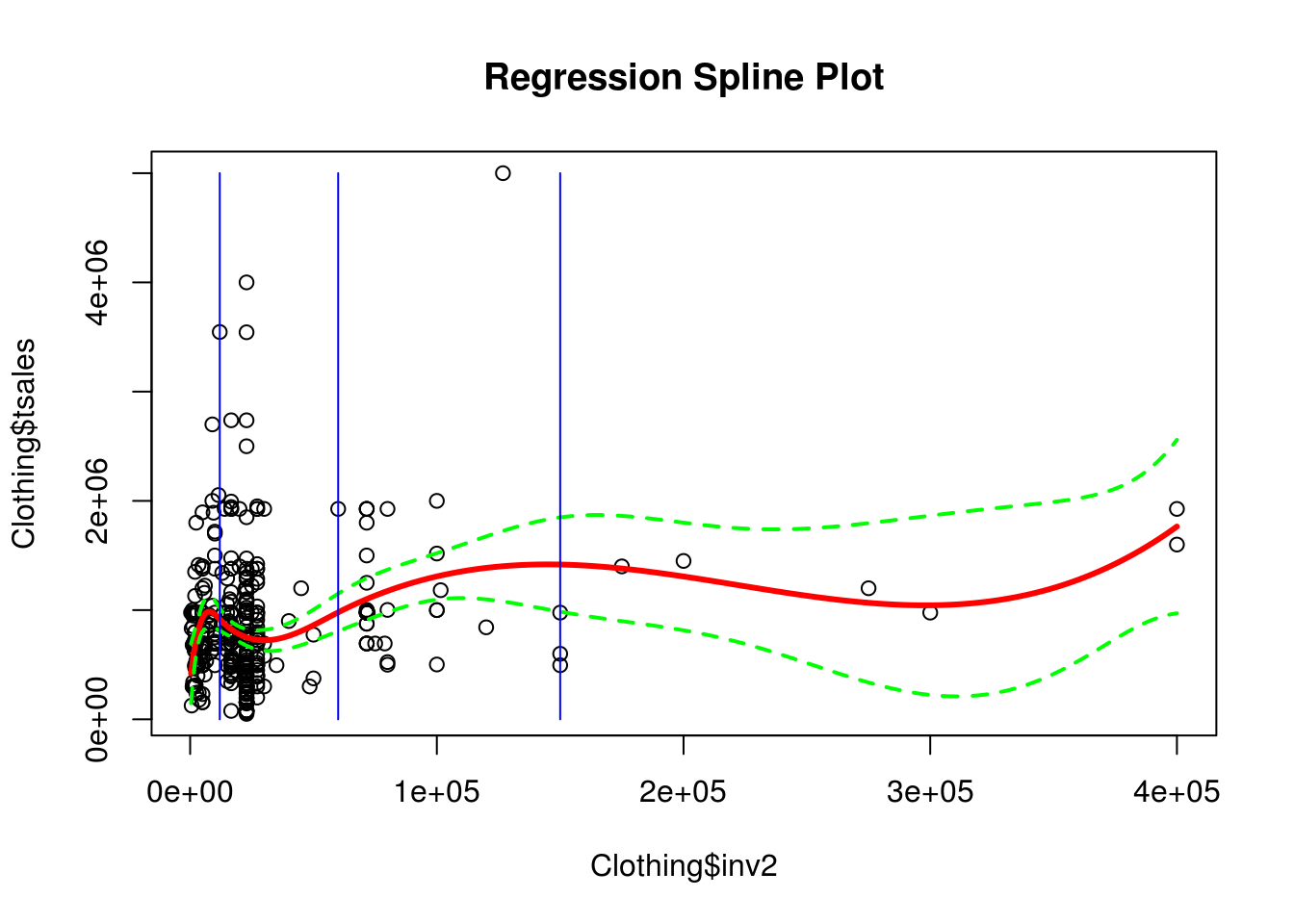When this model was created it was essentially three models connected. Model on goes from the first blue line to the second. Model 2 goes form the second blue line to the third and model three was from the third blue line until the end. This kind of flexibility is valuable in understanding  nonlinear relationship

Factors in R

# Logistic Polynomial Regression in R

Polynomial regression is used when you want to develop a regression model that is not linear. It is common to use this method when performing traditional least squares regression. However, it is also possible to use polynomial regression when the dependent variable is categorical. As such, in this post, we will go through an example of logistic polynomial regression.

Specifically, we will use the “Clothing” dataset from the “Ecdat” package. We will divide the “tsales” dependent variable into two categories to run the analysis. Below is the code to get started.

``library(Ecdat)``
``data(Clothing)``

There is little preparation for this example. Below is the code for the model

``fitglm<-glm(I(tsales>900000)~poly(inv2,4),data=Clothing,family = binomial)``

Here is what we did

1. We created an object called “fitglm” to save our results
2. We used the “glm” function to process the model
3. We used the “I” function. This told R to process the information inside the parentheses as is. As such, we did not have to make a new variable in which we split the “tsales” variable. Simply, if sales were greater than 900000 it was code 1 and 0 if less than this amount.
4. Next, we set the information for the independent variable. We used the “poly” function. Inside this function, we placed the “inv2” variable and the highest order polynomial we want to explore.
5. We set the data to “Clothing”
6. Lastly, we set the “family” argument to “binomial” which is needed for logistic regression

Below is the results

``summary(fitglm)``
``````##
## Call:
## glm(formula = I(tsales > 9e+05) ~ poly(inv2, 4), family = binomial,
##     data = Clothing)
##
## Deviance Residuals:
##     Min       1Q   Median       3Q      Max
## -1.5025  -0.8778  -0.8458   1.4534   1.5681
##
## Coefficients:
##                Estimate Std. Error z value Pr(>|z|)
## (Intercept)       3.074      2.685   1.145   0.2523
## poly(inv2, 4)1  641.710    459.327   1.397   0.1624
## poly(inv2, 4)2  585.975    421.723   1.389   0.1647
## poly(inv2, 4)3  259.700    178.081   1.458   0.1448
## poly(inv2, 4)4   73.425     44.206   1.661   0.0967 .
## ---
## Signif. codes:  0 '***' 0.001 '**' 0.01 '*' 0.05 '.' 0.1 ' ' 1
##
## (Dispersion parameter for binomial family taken to be 1)
##
##     Null deviance: 521.57  on 399  degrees of freedom
## Residual deviance: 493.51  on 395  degrees of freedom
## AIC: 503.51
##
## Number of Fisher Scoring iterations: 13``````

It appears that only the 4th-degree polynomial is significant and barely at that. We will now find the range of our independent variable “inv2” and make a grid from this information. Doing this will allow us to run our model using the full range of possible values for our independent variable.

``````inv2lims<-range(Clothing\$inv2)
inv2.grid<-seq(from=inv2lims,to=inv2lims)``````

The “inv2lims” object has two values. The lowest value in “inv2” and the highest value. These values serve as the highest and lowest values in our “inv2.grid” object. This means that we have values started at 350 and going to 400000 by 1 in a grid to be used as values for “inv2” in our prediction model. Below is our prediction model.

``predsglm<-predict(fitglm,newdata=list(inv2=inv2.grid),se=T,type="response")``

Next, we need to calculate the probabilities that a given value of “inv2” predicts a store has “tsales” greater than 900000. The equation is as follows.

``pfit<-exp(predsglm\$fit)/(1+exp(predsglm\$fit))``

Graphing this leads to interesting insights. Below is the code

``plot(pfit)``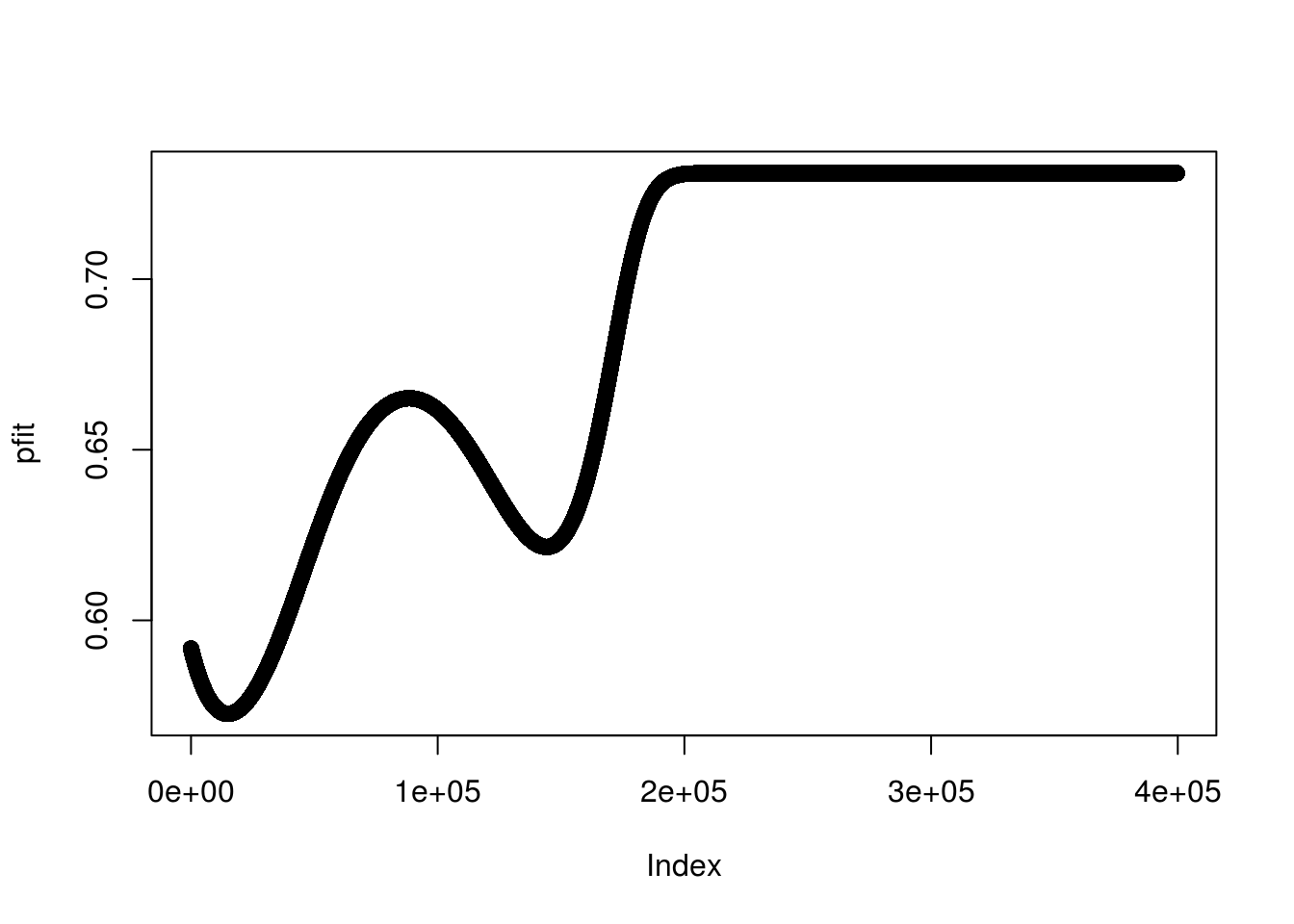You can see the curves in the line from the polynomial expression. As it appears. As inv2 increase the probability increase until the values fall between 125000 and 200000. This is interesting, to say the least.

We now need to plot the actual model. First, we need to calculate the confidence intervals. This is done with the code below.

``````se.bandsglm.logit<-cbind(predsglm\$fit+2*predsglm\$se.fit,predsglm\$fit-2*predsglm\$se.fit)
se.bandsglm<-exp(se.bandsglm.logit)/(1+exp(se.bandsglm.logit))``````

The ’se.bandsglm” object contains the log odds of each example and the “se.bandsglm” has the probabilities. Now we plot the results

``````plot(Clothing\$inv2,I(Clothing\$tsales>900000),xlim=inv2lims,type='n')
points(jitter(Clothing\$inv2),I((Clothing\$tsales>900000)),cex=2,pch='|',col='darkgrey')
lines(inv2.grid,pfit,lwd=4)
matlines(inv2.grid,se.bandsglm,col="green",lty=6,lwd=6)``````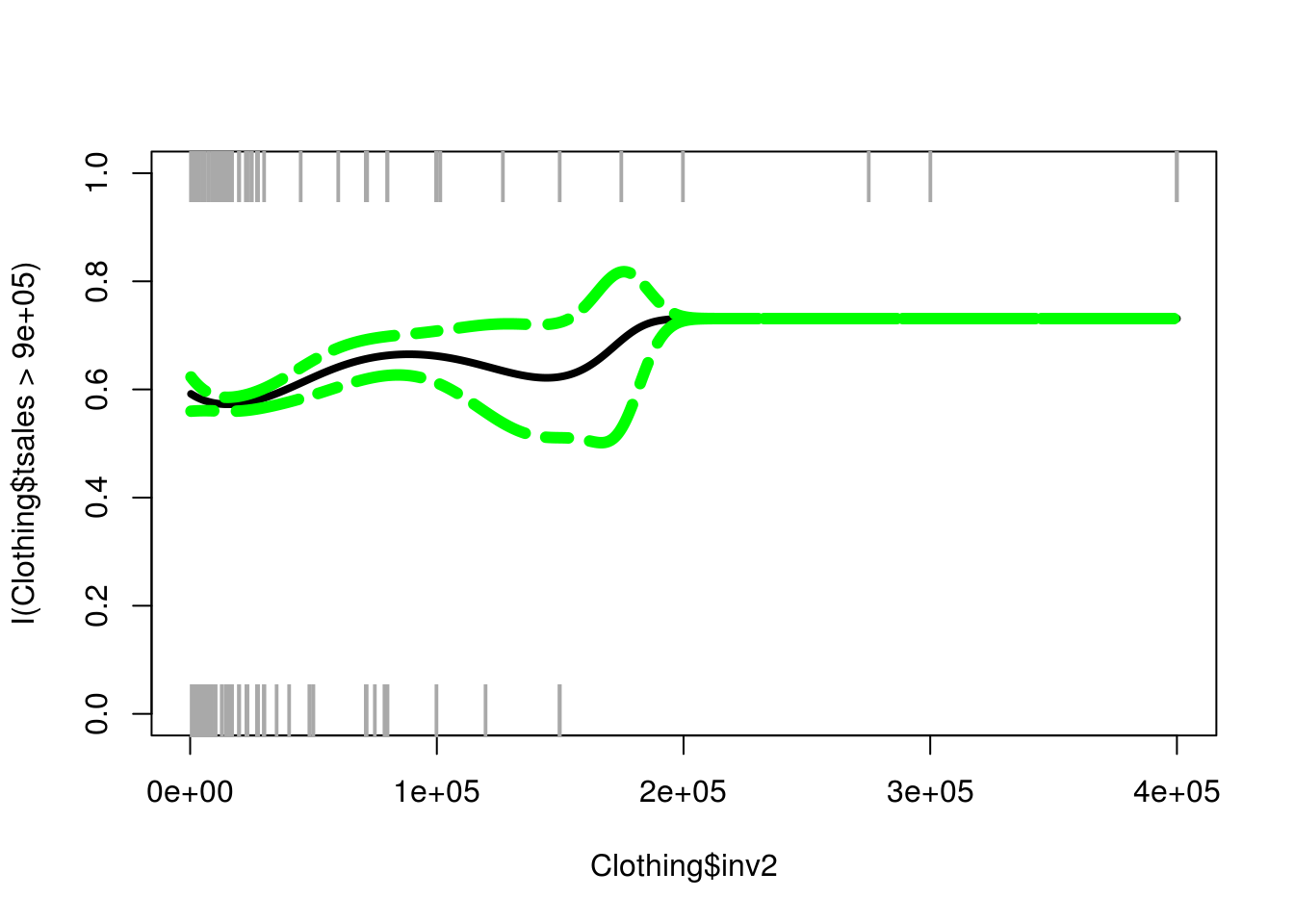In the code above we did the following.
1. We plotted our dependent and independent variables. However, we set the argument “type” to n which means nothing. This was done so we can add the information step-by-step.
2. We added the points. This was done using the “points” function. The “jitter” function just helps to spread the information out. The other arguments (cex, pch, col) our for aesthetics and our optional.
3. We add our logistic polynomial line based on our independent variable grid and the “pfit” object which has all of the predicted probabilities.
4. Last, we add the confidence intervals using the “matlines” function. Which includes the grid object as well as the “se.bandsglm” information.

You can see that these results are similar to when we only graphed the “pfit” information. However, we also add the confidence intervals. You can see the same dip around 125000-200000 were there is also a larger confidence interval. if you look at the plot you can see that there are fewer data points in this range which may be what is making the intervals wider.

Conclusion

Logistic polynomial regression allows the regression line to have more curves to it if it is necessary. This is useful for fitting data that is non-linear in nature.

# Polynomial Regression in R

Polynomial regression is one of the easiest ways to fit a non-linear line to a data set. This is done through the use of higher order polynomials such as cubic, quadratic, etc to one or more predictor variables in a model.Generally, polynomial regression is used for one predictor and one outcome variable. When there are several predictor variables it is more common to use generalized additive modeling/ In this post, we will use the “Clothing” dataset from the “Ecdat” package to predict total sales with the use of polynomial regression. Below is some initial code.

````library(Ecdat)````
data(Clothing)
str(Clothing)``````
``````## 'data.frame':    400 obs. of  13 variables:
##  \$ tsales : int  750000 1926395 1250000 694227 750000 400000 1300000 495340 1200000 495340 ...
##  \$ sales  : num  4412 4281 4167 2670 15000 ...
##  \$ margin : num  41 39 40 40 44 41 39 28 41 37 ...
##  \$ nown   : num  1 2 1 1 2 ...
##  \$ nfull  : num  1 2 2 1 1.96 ...
##  \$ npart  : num  1 3 2.22 1.28 1.28 ...
##  \$ naux   : num  1.54 1.54 1.41 1.37 1.37 ...
##  \$ hoursw : int  76 192 114 100 104 72 161 80 158 87 ...
##  \$ hourspw: num  16.8 22.5 17.2 21.5 15.7 ...
##  \$ inv1   : num  17167 17167 292857 22207 22207 ...
##  \$ inv2   : num  27177 27177 71571 15000 10000 ...
##  \$ ssize  : int  170 450 300 260 50 90 400 100 450 75 ...
##  \$ start  : num  41 39 40 40 44 41 39 28 41 37 ...``````

We are going to use the “inv2” variable as our predictor. This variable measures the investment in automation by a particular store. We will now run our polynomial regression model.

``````fit<-lm(tsales~poly(inv2,5),data = Clothing)
summary(fit)``````
``````##
## Call:
## lm(formula = tsales ~ poly(inv2, 5), data = Clothing)
##
## Residuals:
##     Min      1Q  Median      3Q     Max
## -946668 -336447  -96763  184927 3599267
##
## Coefficients:
##                Estimate Std. Error t value Pr(>|t|)
## (Intercept)      833584      28489  29.259  < 2e-16 ***
## poly(inv2, 5)1  2391309     569789   4.197 3.35e-05 ***
## poly(inv2, 5)2  -665063     569789  -1.167   0.2438
## poly(inv2, 5)3    49793     569789   0.087   0.9304
## poly(inv2, 5)4  1279190     569789   2.245   0.0253 *
## poly(inv2, 5)5  -341189     569789  -0.599   0.5497
## ---
## Signif. codes:  0 '***' 0.001 '**' 0.01 '*' 0.05 '.' 0.1 ' ' 1
##
## Residual standard error: 569800 on 394 degrees of freedom
## Multiple R-squared:  0.05828,    Adjusted R-squared:  0.04633
## F-statistic: 4.876 on 5 and 394 DF,  p-value: 0.0002428``````

The code above should be mostly familiar. We use the “lm” function as normal for regression. However, we then used the “poly” function on the “inv2” variable. What this does is runs our model 5 times (5 is the number next to “inv2”). Each time a different polynomial is used from 1 (no polynomial) to 5 (5th order polynomial). The results indicate that the 4th-degree polynomial is significant.

We now will prepare a visual of the results but first, there are several things we need to prepare. First, we want to find what the range of our predictor variable “inv2” is and we will save this information in a grade. The code is below.

``inv2lims<-range(Clothing\$inv2)``

Second, we need to create a grid that has all the possible values of “inv2” from the lowest to the highest broken up in intervals of one. Below is the code.

``inv2.grid<-seq(from=inv2lims,to=inv2lims)``

We now have a dataset with almost 400000 data points in the “inv2.grid” object through this approach. We will now use these values to predict “tsales.” We also want the standard errors so we se “se” to TRUE

``preds<-predict(fit,newdata=list(inv2=inv2.grid),se=TRUE)``

We now need to find the confidence interval for our regression line. This is done by making a dataframe that takes the predicted fit adds or subtracts 2 and multiples this number by the standard error as shown below.

``se.bands<-cbind(preds\$fit+2*preds\$se.fit,preds\$fit-2*preds\$se.fit)``

With these steps completed, we are ready to create our civilization.

To make our visual, we use the “plot” function on the predictor and outcome. Doing this gives us a plot without a regression line. We then use the “lines” function to add the polynomial regression line, however, this line is based on the “inv2.grid” object (40,000 observations) and our predictions. Lastly, we use the “matlines” function to add the confidence intervals we found and stored in the “se.bands” object.

``````plot(Clothing\$inv2,Clothing\$tsales)
lines(inv2.grid,preds\$fit,lwd=4,col='blue')
matlines(inv2.grid,se.bands,lwd = 4,col = "yellow",lty=4)``````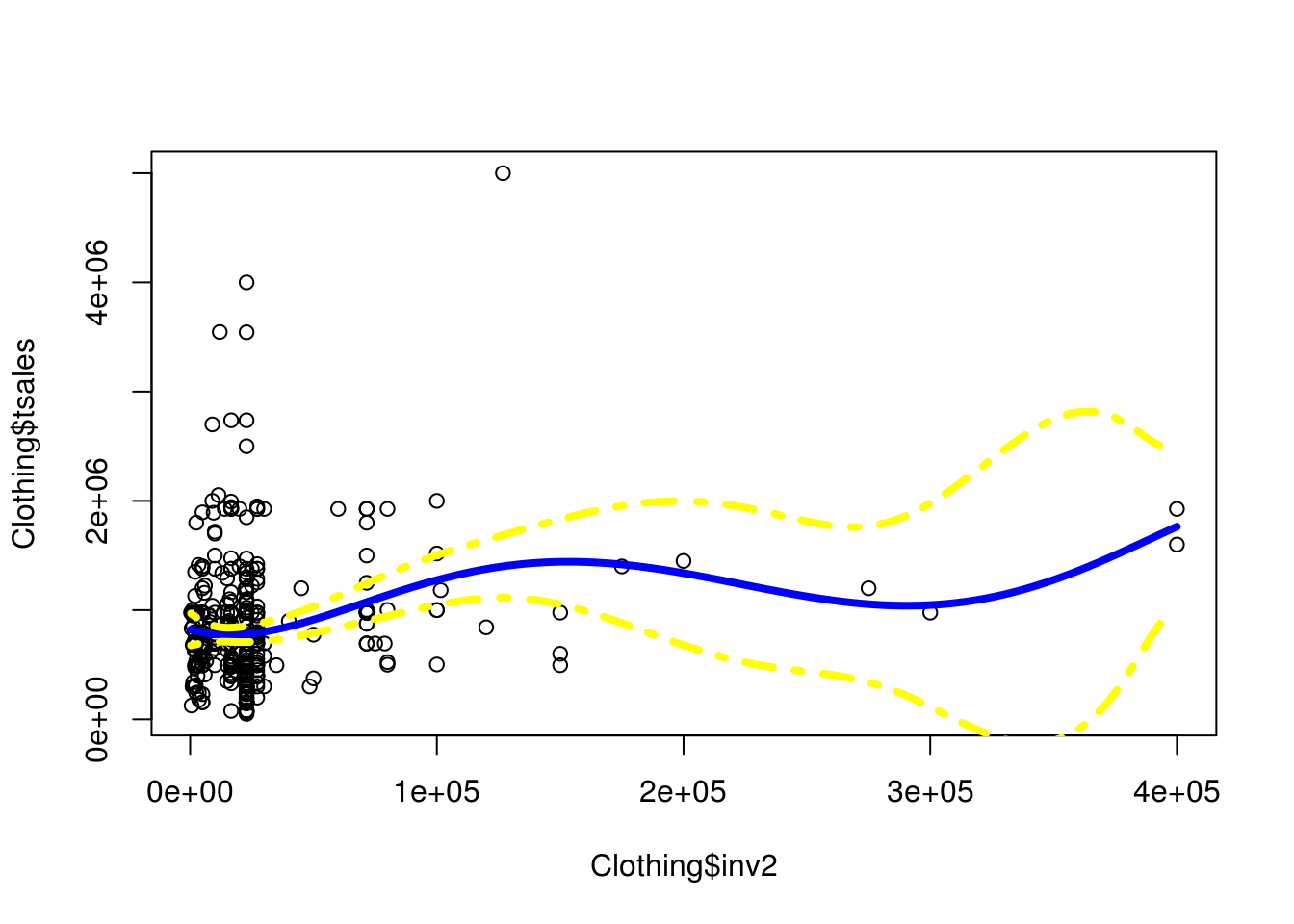Conclusion

You can clearly see the curvature of the line. Which helped to improve model fit. Now any of you can tell that we are fitting this line to mostly outliers. This is one reason we the standard error gets wider and wider it is because there are fewer and fewer observations on which to base it. However, for demonstration purposes, this is a clear example of the power of polynomial regression.

# Search Text within Vector in R VIDEO

Searching text within an object in R

# Partial Least Squares Regression in R

Partial least squares regression is a form of regression that involves the development of components of the original variables in a supervised way. Whatthis means is that the dependent variable is used to help create the new components form the original variables. This means that when pls is used the linear combination of the new features helps to explain both the independent and dependent variables in the model.

In this post, we will use predict “income” in the “Mroz” dataset using pls. Below is some initial code.

``library(pls);library(Ecdat)``
``````data("Mroz")
str(Mroz)``````
``````## 'data.frame':    753 obs. of  18 variables:
##  \$ work      : Factor w/ 2 levels "yes","no": 2 2 2 2 2 2 2 2 2 2 ...
##  \$ hoursw    : int  1610 1656 1980 456 1568 2032 1440 1020 1458 1600 ...
##  \$ child6    : int  1 0 1 0 1 0 0 0 0 0 ...
##  \$ child618  : int  0 2 3 3 2 0 2 0 2 2 ...
##  \$ agew      : int  32 30 35 34 31 54 37 54 48 39 ...
##  \$ educw     : int  12 12 12 12 14 12 16 12 12 12 ...
##  \$ hearnw    : num  3.35 1.39 4.55 1.1 4.59 ...
##  \$ wagew     : num  2.65 2.65 4.04 3.25 3.6 4.7 5.95 9.98 0 4.15 ...
##  \$ hoursh    : int  2708 2310 3072 1920 2000 1040 2670 4120 1995 2100 ...
##  \$ ageh      : int  34 30 40 53 32 57 37 53 52 43 ...
##  \$ educh     : int  12 9 12 10 12 11 12 8 4 12 ...
##  \$ wageh     : num  4.03 8.44 3.58 3.54 10 ...
##  \$ income    : int  16310 21800 21040 7300 27300 19495 21152 18900 20405 20425 ...
##  \$ educwm    : int  12 7 12 7 12 14 14 3 7 7 ...
##  \$ educwf    : int  7 7 7 7 14 7 7 3 7 7 ...
##  \$ unemprate : num  5 11 5 5 9.5 7.5 5 5 3 5 ...
##  \$ city      : Factor w/ 2 levels "no","yes": 1 2 1 1 2 2 1 1 1 1 ...
##  \$ experience: int  14 5 15 6 7 33 11 35 24 21 ...``````

First, we must prepare our data by dividing it into a training and test set. We will do this by doing a 50/50 split of the data.

``````set.seed(777)
train<-sample(c(T,F),nrow(Mroz),rep=T) #50/50 train/test split
test<-(!train)``````

In the code above we set the “set.seed function in order to assure reduplication. Then we created the “train” object and used the “sample” function to make a vector with ‘T’ and ‘F’ based on the number of rows in “Mroz”. Lastly, we created the “test”” object base don everything that is not in the “train” object as that is what the exclamation point is for.

Now we create our model using the “plsr” function from the “pls” package and we will examine the results using the “summary” function. We will also scale the data since this the scale affects the development of the components and use cross-validation. Below is the code.

``````set.seed(777)
pls.fit<-plsr(income~.,data=Mroz,subset=train,scale=T,validation="CV")
summary(pls.fit)``````
``````## Data:    X dimension: 392 17
##  Y dimension: 392 1
## Fit method: kernelpls
## Number of components considered: 17
##
## VALIDATION: RMSEP
## Cross-validated using 10 random segments.
##        (Intercept)  1 comps  2 comps  3 comps  4 comps  5 comps  6 comps
## CV           11218     8121     6701     6127     5952     5886     5857
## adjCV        11218     8114     6683     6108     5941     5872     5842
##        7 comps  8 comps  9 comps  10 comps  11 comps  12 comps  13 comps
## CV        5853     5849     5854      5853      5853      5852      5852
## adjCV     5837     5833     5837      5836      5836      5835      5835
##        14 comps  15 comps  16 comps  17 comps
## CV         5852      5852      5852      5852
## adjCV      5835      5835      5835      5835
##
## TRAINING: % variance explained
##         1 comps  2 comps  3 comps  4 comps  5 comps  6 comps  7 comps
## X         17.04    26.64    37.18    49.16    59.63    64.63    69.13
## income    49.26    66.63    72.75    74.16    74.87    75.25    75.44
##         8 comps  9 comps  10 comps  11 comps  12 comps  13 comps  14 comps
## X         72.82    76.06     78.59     81.79     85.52     89.55     92.14
## income    75.49    75.51     75.51     75.52     75.52     75.52     75.52
##         15 comps  16 comps  17 comps
## X          94.88     97.62    100.00
## income     75.52     75.52     75.52``````

The printout includes the root mean squared error for each of the components in the VALIDATION section as well as the variance explained in the TRAINING section. There are 17 components because there are 17 independent variables. You can see that after component 3 or 4 there is little improvement in the variance explained in the dependent variable. Below is the code for the plot of these results. It requires the use of the “validationplot” function with the “val.type” argument set to “MSEP” Below is the code

``validationplot(pls.fit,val.type = "MSEP")``We will do the predictions with our model. We use the “predict” function, use our “Mroz” dataset but only those index in the “test” vector and set the components to three based on our previous plot. Below is the code.

``````set.seed(777)
pls.pred<-predict(pls.fit,Mroz[test,],ncomp=3)``````

After this, we will calculate the mean squared error. This is done by subtracting the results of our predicted model from the dependent variable of the test set. We then square this information and calculate the mean. Below is the code

``mean((pls.pred-Mroz\$income[test])^2)``
``##  63386682``

As you know, this information is only useful when compared to something else. Therefore, we will run the data with a tradition least squares regression model and compare the results.

``````set.seed(777)
lm.fit<-lm(income~.,data=Mroz,subset=train)
lm.pred<-predict(lm.fit,Mroz[test,])
mean((lm.pred-Mroz\$income[test])^2)``````
``##  59432814``

The least squares model is slightly better then our partial least squares model but if we look at the model we see several variables that are not significant. We will remove these see what the results are

``summary(lm.fit)``
``````##
## Call:
## lm(formula = income ~ ., data = Mroz, subset = train)
##
## Residuals:
##    Min     1Q Median     3Q    Max
## -20131  -2923  -1065   1670  36246
##
## Coefficients:
##               Estimate Std. Error t value Pr(>|t|)
## (Intercept) -1.946e+04  3.224e+03  -6.036 3.81e-09 ***
## workno      -4.823e+03  1.037e+03  -4.651 4.59e-06 ***
## hoursw       4.255e+00  5.517e-01   7.712 1.14e-13 ***
## child6      -6.313e+02  6.694e+02  -0.943 0.346258
## child618     4.847e+02  2.362e+02   2.052 0.040841 *
## agew         2.782e+02  8.124e+01   3.424 0.000686 ***
## educw        1.268e+02  1.889e+02   0.671 0.502513
## hearnw       6.401e+02  1.420e+02   4.507 8.79e-06 ***
## wagew        1.945e+02  1.818e+02   1.070 0.285187
## hoursh       6.030e+00  5.342e-01  11.288  < 2e-16 ***
## ageh        -9.433e+01  7.720e+01  -1.222 0.222488
## educh        1.784e+02  1.369e+02   1.303 0.193437
## wageh        2.202e+03  8.714e+01  25.264  < 2e-16 ***
## educwm      -4.394e+01  1.128e+02  -0.390 0.697024
## educwf       1.392e+02  1.053e+02   1.322 0.186873
## unemprate   -1.657e+02  9.780e+01  -1.694 0.091055 .
## cityyes     -3.475e+02  6.686e+02  -0.520 0.603496
## experience  -1.229e+02  4.490e+01  -2.737 0.006488 **
## ---
## Signif. codes:  0 '***' 0.001 '**' 0.01 '*' 0.05 '.' 0.1 ' ' 1
##
## Residual standard error: 5668 on 374 degrees of freedom
## Multiple R-squared:  0.7552, Adjusted R-squared:  0.744
## F-statistic: 67.85 on 17 and 374 DF,  p-value: < 2.2e-16``````
``````set.seed(777)
lm.fit<-lm(income~work+hoursw+child618+agew+hearnw+hoursh+wageh+experience,data=Mroz,subset=train)
lm.pred<-predict(lm.fit,Mroz[test,])
mean((lm.pred-Mroz\$income[test])^2)``````
``##  57839715``

As you can see the error decreased even more which indicates that the least squares regression model is superior to the partial least squares model. In addition, the partial least squares model is much more difficult to explain because of the use of components. As such, the least squares model is the favored one.

# Principal Component Regression in R

This post will explain and provide an example of principal component regression (PCR).Principal component regression involves having the model construct components from the independent variables that are a linear combination of the independent variables. This is similar to principal component analysis but the components are designed in a way to best explain the dependent variable. Doing this often allows you to use fewer variables in your model and usually improves the fit of your model as well.

Since PCR is based on principal component analysis it is an unsupervised method, which means the dependent variable has no influence on the development of the components. As such, there are times when the components that are developed may not be beneficial for explaining the dependent variable.

Our example will use the “Mroz” dataset from the “Ecdat” package. Our goal will be to predict “income” based on the variables in the dataset. Below is the initial code

``library(pls);library(Ecdat)``
``````data(Mroz)
str(Mroz)``````
``````## 'data.frame':    753 obs. of  18 variables:
##  \$ work      : Factor w/ 2 levels "yes","no": 2 2 2 2 2 2 2 2 2 2 ...
##  \$ hoursw    : int  1610 1656 1980 456 1568 2032 1440 1020 1458 1600 ...
##  \$ child6    : int  1 0 1 0 1 0 0 0 0 0 ...
##  \$ child618  : int  0 2 3 3 2 0 2 0 2 2 ...
##  \$ agew      : int  32 30 35 34 31 54 37 54 48 39 ...
##  \$ educw     : int  12 12 12 12 14 12 16 12 12 12 ...
##  \$ hearnw    : num  3.35 1.39 4.55 1.1 4.59 ...
##  \$ wagew     : num  2.65 2.65 4.04 3.25 3.6 4.7 5.95 9.98 0 4.15 ...
##  \$ hoursh    : int  2708 2310 3072 1920 2000 1040 2670 4120 1995 2100 ...
##  \$ ageh      : int  34 30 40 53 32 57 37 53 52 43 ...
##  \$ educh     : int  12 9 12 10 12 11 12 8 4 12 ...
##  \$ wageh     : num  4.03 8.44 3.58 3.54 10 ...
##  \$ income    : int  16310 21800 21040 7300 27300 19495 21152 18900 20405 20425 ...
##  \$ educwm    : int  12 7 12 7 12 14 14 3 7 7 ...
##  \$ educwf    : int  7 7 7 7 14 7 7 3 7 7 ...
##  \$ unemprate : num  5 11 5 5 9.5 7.5 5 5 3 5 ...
##  \$ city      : Factor w/ 2 levels "no","yes": 1 2 1 1 2 2 1 1 1 1 ...
##  \$ experience: int  14 5 15 6 7 33 11 35 24 21 ...``````

Our first step is to divide our dataset into a train and test set. We will do a simple 50/50 split for this demonstration.

``````train<-sample(c(T,F),nrow(Mroz),rep=T) #50/50 train/test split
test<-(!train)``````

In the code above we use the “sample” function to create a “train” index based on the number of rows in the “Mroz” dataset. Basically, R is making a vector that randomly assigns different rows in the “Mroz” dataset to be marked as True or False. Next, we use the “train” vector and we assign everything or every number that is not in the “train” vector to the test vector by using the exclamation mark.

We are now ready to develop our model. Below is the code

``````set.seed(777)
pcr.fit<-pcr(income~.,data=Mroz,subset=train,scale=T,validation="CV")``````

To make our model we use the “pcr” function from the “pls” package. The “subset” argument tells r to use the “train” vector to select examples from the “Mroz” dataset. The “scale” argument makes sure everything is measured the same way. This is important when using a component analysis tool as variables with different scale have a different influence on the components. Lastly, the “validation” argument enables cross-validation. This will help us to determine the number of components to use for prediction. Below is the results of the model using the “summary” function.

``summary(pcr.fit)``
``````## Data:    X dimension: 381 17
##  Y dimension: 381 1
## Fit method: svdpc
## Number of components considered: 17
##
## VALIDATION: RMSEP
## Cross-validated using 10 random segments.
##        (Intercept)  1 comps  2 comps  3 comps  4 comps  5 comps  6 comps
## CV           12102    11533    11017     9863     9884     9524     9563
## adjCV        12102    11534    11011     9855     9878     9502     9596
##        7 comps  8 comps  9 comps  10 comps  11 comps  12 comps  13 comps
## CV        9149     9133     8811      8527      7265      7234      7120
## adjCV     9126     9123     8798      8877      7199      7172      7100
##        14 comps  15 comps  16 comps  17 comps
## CV         7118      7141      6972      6992
## adjCV      7100      7123      6951      6969
##
## TRAINING: % variance explained
##         1 comps  2 comps  3 comps  4 comps  5 comps  6 comps  7 comps
## X        21.359    38.71    51.99    59.67    65.66    71.20    76.28
## income    9.927    19.50    35.41    35.63    41.28    41.28    46.75
##         8 comps  9 comps  10 comps  11 comps  12 comps  13 comps  14 comps
## X         80.70    84.39     87.32     90.15     92.65     95.02     96.95
## income    47.08    50.98     51.73     68.17     68.29     68.31     68.34
##         15 comps  16 comps  17 comps
## X          98.47     99.38    100.00
## income     68.48     70.29     70.39``````

There is a lot of information here.The VALIDATION: RMSEP section gives you the root mean squared error of the model broken down by component. The TRAINING section is similar the printout of any PCA but it shows the amount of cumulative variance of the components, as well as the variance, explained for the dependent variable “income.” In this model, we are able to explain up to 70% of the variance if we use all 17 components.

We can graph the MSE using the “validationplot” function with the argument “val.type” set to “MSEP”. The code is below.

``validationplot(pcr.fit,val.type = "MSEP")``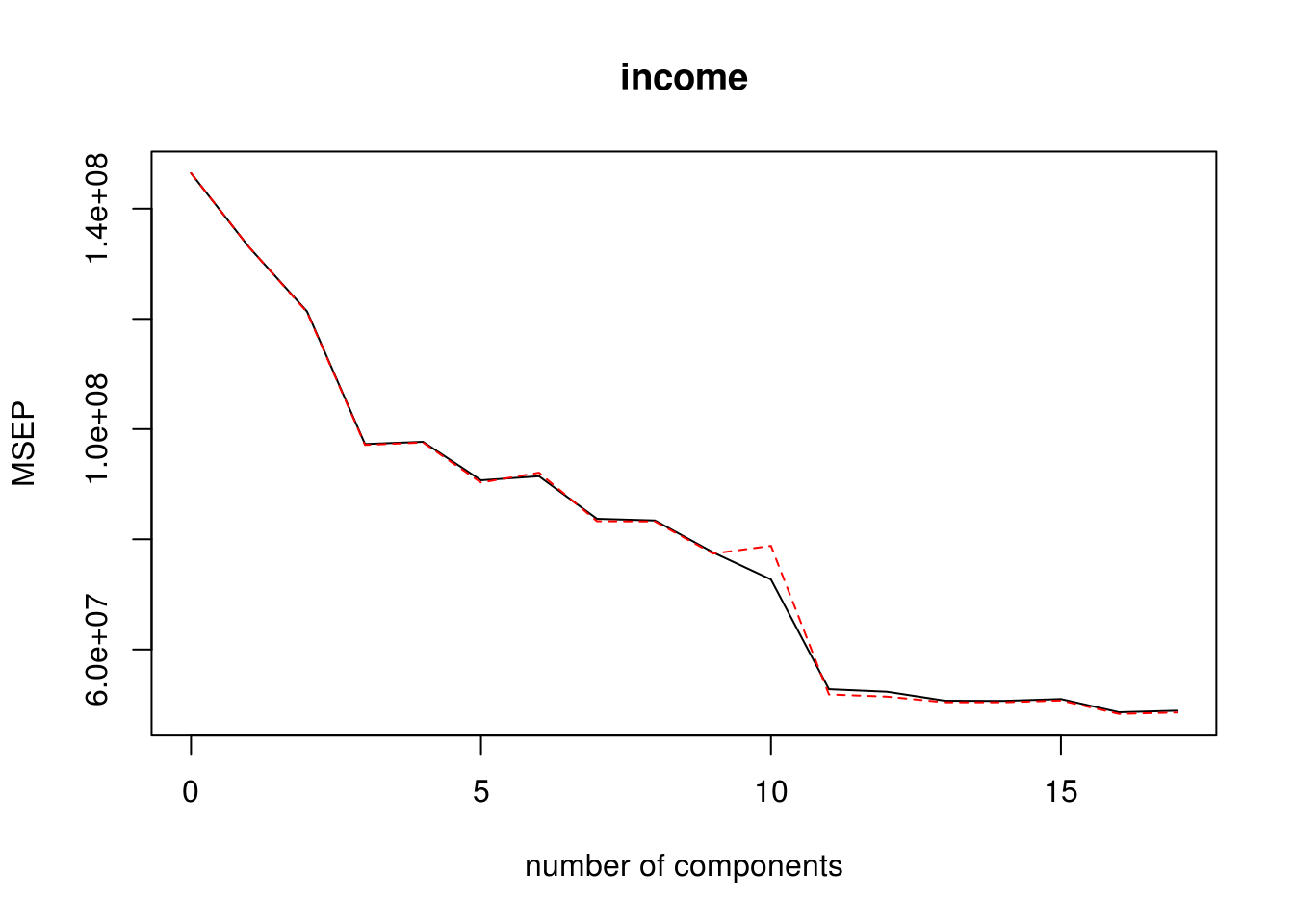How many components to pick is subjective, however, there is almost no improvement beyond 13 so we will use 13 components in our prediction model and we will calculate the means squared error.

``````set.seed(777)
pcr.pred<-predict(pcr.fit,Mroz[test,],ncomp=13)
mean((pcr.pred-Mroz\$income[test])^2)``````
``##  48958982``

MSE is what you would use to compare this model to other models that you developed. Below is the performance of a least squares regression model

``````set.seed(777)
lm.fit<-lm(income~.,data=Mroz,subset=train)
lm.pred<-predict(lm.fit,Mroz[test,])
mean((lm.pred-Mroz\$income[test])^2)``````
``##  47794472``

If you compare the MSE the least squares model performs slightly better than the PCR one. However, there are a lot of non-significant features in the model as shown below.

``summary(lm.fit)``
``````##
## Call:
## lm(formula = income ~ ., data = Mroz, subset = train)
##
## Residuals:
##    Min     1Q Median     3Q    Max
## -27646  -3337  -1387   1860  48371
##
## Coefficients:
##               Estimate Std. Error t value Pr(>|t|)
## (Intercept) -2.215e+04  3.987e+03  -5.556 5.35e-08 ***
## workno      -3.828e+03  1.316e+03  -2.909  0.00385 **
## hoursw       3.955e+00  7.085e-01   5.582 4.65e-08 ***
## child6       5.370e+02  8.241e+02   0.652  0.51512
## child618     4.250e+02  2.850e+02   1.491  0.13673
## agew         1.962e+02  9.849e+01   1.992  0.04709 *
## educw        1.097e+02  2.276e+02   0.482  0.63013
## hearnw       9.835e+02  2.303e+02   4.270 2.50e-05 ***
## wagew        2.292e+02  2.423e+02   0.946  0.34484
## hoursh       6.386e+00  6.144e-01  10.394  < 2e-16 ***
## ageh        -1.284e+01  9.762e+01  -0.132  0.89542
## educh        1.460e+02  1.592e+02   0.917  0.35982
## wageh        2.083e+03  9.930e+01  20.978  < 2e-16 ***
## educwm       1.354e+02  1.335e+02   1.014  0.31115
## educwf       1.653e+02  1.257e+02   1.315  0.18920
## unemprate   -1.213e+02  1.148e+02  -1.057  0.29140
## cityyes     -2.064e+02  7.905e+02  -0.261  0.79421
## experience  -1.165e+02  5.393e+01  -2.159  0.03147 *
## ---
## Signif. codes:  0 '***' 0.001 '**' 0.01 '*' 0.05 '.' 0.1 ' ' 1
##
## Residual standard error: 6729 on 363 degrees of freedom
## Multiple R-squared:  0.7039, Adjusted R-squared:   0.69
## F-statistic: 50.76 on 17 and 363 DF,  p-value: < 2.2e-16``````

Removing these and the MSE is almost the same for the PCR and least square models

``````set.seed(777)
lm.fit2<-lm(income~work+hoursw+hearnw+hoursh+wageh,data=Mroz,subset=train)
lm.pred2<-predict(lm.fit2,Mroz[test,])
mean((lm.pred2-Mroz\$income[test])^2)``````
``##  47968996``

Conclusion

Since the least squares model is simpler it is probably the superior model. PCR is strongest when there are a lot of variables involve and if there are issues with multicollinearity.

# Combining and Splitting Strings in R VIDEO

Combining and Splitting Strings in R

# Example of Best Subset Regression in R

This post will provide an example of best subset regression. This is a topicthat has been covered before in this blog. However, in the current post, we will approach this using a slightly different coding and a different dataset. We will be using the “HI” dataset from the “Ecdat” package. Our goal will be to predict the number of hours a women works based on the other variables in the dataset. Below is some initial code.

``library(leaps);library(Ecdat)``
``````data(HI)
str(HI)``````
``````## 'data.frame':    22272 obs. of  13 variables:
##  \$ whrswk    : int  0 50 40 40 0 40 40 25 45 30 ...
##  \$ hhi       : Factor w/ 2 levels "no","yes": 1 1 2 1 2 2 2 1 1 1 ...
##  \$ whi       : Factor w/ 2 levels "no","yes": 1 2 1 2 1 2 1 1 2 1 ...
##  \$ hhi2      : Factor w/ 2 levels "no","yes": 1 1 2 2 2 2 2 1 1 2 ...
##  \$ education : Ord.factor w/ 6 levels "<9years"<"9-11years"<..: 4 4 3 4 2 3 5 3 5 4 ...
##  \$ race      : Factor w/ 3 levels "white","black",..: 1 1 1 1 1 1 1 1 1 1 ...
##  \$ hispanic  : Factor w/ 2 levels "no","yes": 1 1 1 1 1 1 1 1 1 1 ...
##  \$ experience: num  13 24 43 17 44.5 32 14 1 4 7 ...
##  \$ kidslt6   : int  2 0 0 0 0 0 0 1 0 1 ...
##  \$ kids618   : int  1 1 0 1 0 0 0 0 0 0 ...
##  \$ husby     : num  12 1.2 31.3 9 0 ...
##  \$ region    : Factor w/ 4 levels "other","northcentral",..: 2 2 2 2 2 2 2 2 2 2 ...
##  \$ wght      : int  214986 210119 219955 210317 219955 208148 213615 181960 214874 214874 ...``````

To develop a model we use the “regsubset” function from the “leap” package. Most of the coding is the same as linear regression. The only difference is the “nvmax” argument which is set to 13. The default setting for “nvmax” is 8. This is good if you only have 8 variables. However, the results from the “str” function indicate that we have 13 functions. Therefore, we need to set the “nvmax” argument to 13 instead of the default value of 8 in order to be sure to include all variables. Below is the code

``regfit.full<-regsubsets(whrswk~.,HI, nvmax = 13)``

We can look at the results with the “summary” function. For space reasons, the code is shown but the results will not be shown here.

``summary(regfit.full)``

If you run the code above in your computer you will 13 columns that are named after the variables created. A star in a column means that that variable is included in the model. To the left is the numbers 1-13 which. One means one variable in the model two means two variables in the model etc.

Our next step is to determine which of these models is the best. First, we need to decide what our criteria for inclusion will be. Below is a list of available fit indices.

``names(summary(regfit.full))``
``##  "which"  "rsq"    "rss"    "adjr2"  "cp"     "bic"    "outmat" "obj"``

For our purposes, we will use “rsq” (r-square) and “bic” “Bayesian Information Criteria.” In the code below we are going to save the values for these two fit indices in their own objects.

``````rsq<-summary(regfit.full)\$rsq
bic<-summary(regfit.full)\$bic``````

Now let’s plot them

``plot(rsq,type='l',main="R-Square",xlab="Number of Variables")``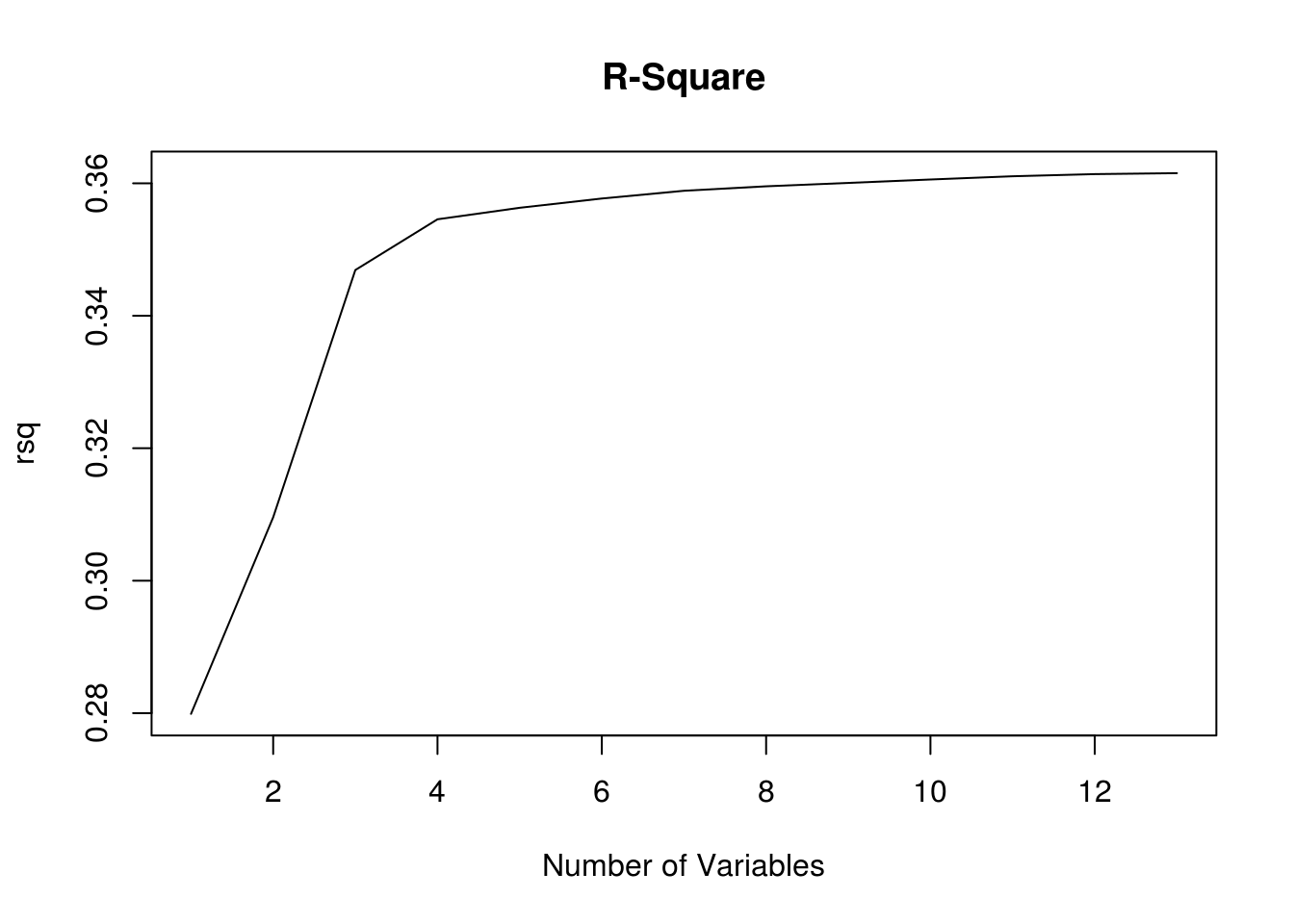``plot(bic,type='l',main="BIC",xlab="Number of Variables")``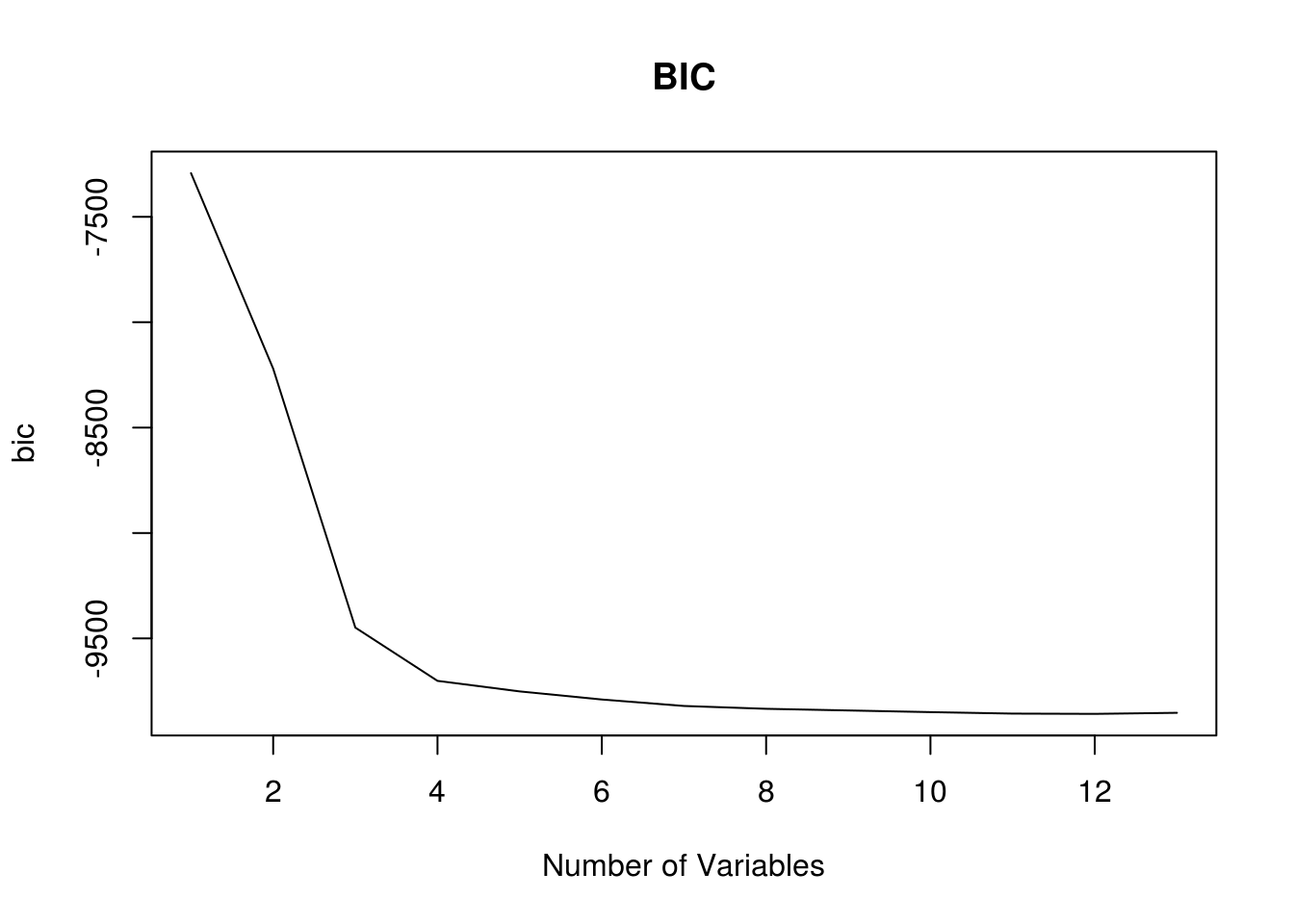You can see that for r-square the values increase and for BIC the values decrease. We will now make both of these plots again but we will have r tell the optimal number of variables when considering each model index. For we use the “which” function to determine the max r-square and the minimum BIC

``which.max(rsq)``
``##  13``
``which.min(bic)``
``##  12``

The model with the best r-square is the one with 13 variables. This makes sense as r-square always improves as you add variables. Since this is a demonstration we will not correct for this. For BIC the lowest values was for 12 variables. We will now plot this information and highlight the best model in the plot using the “points” function, which allows you to emphasis one point in a graph

``````plot(rsq,type='l',main="R-Square with Best Model Highlighted",xlab="Number of Variables")
points(13,(rsq),col="blue",cex=7,pch=20)``````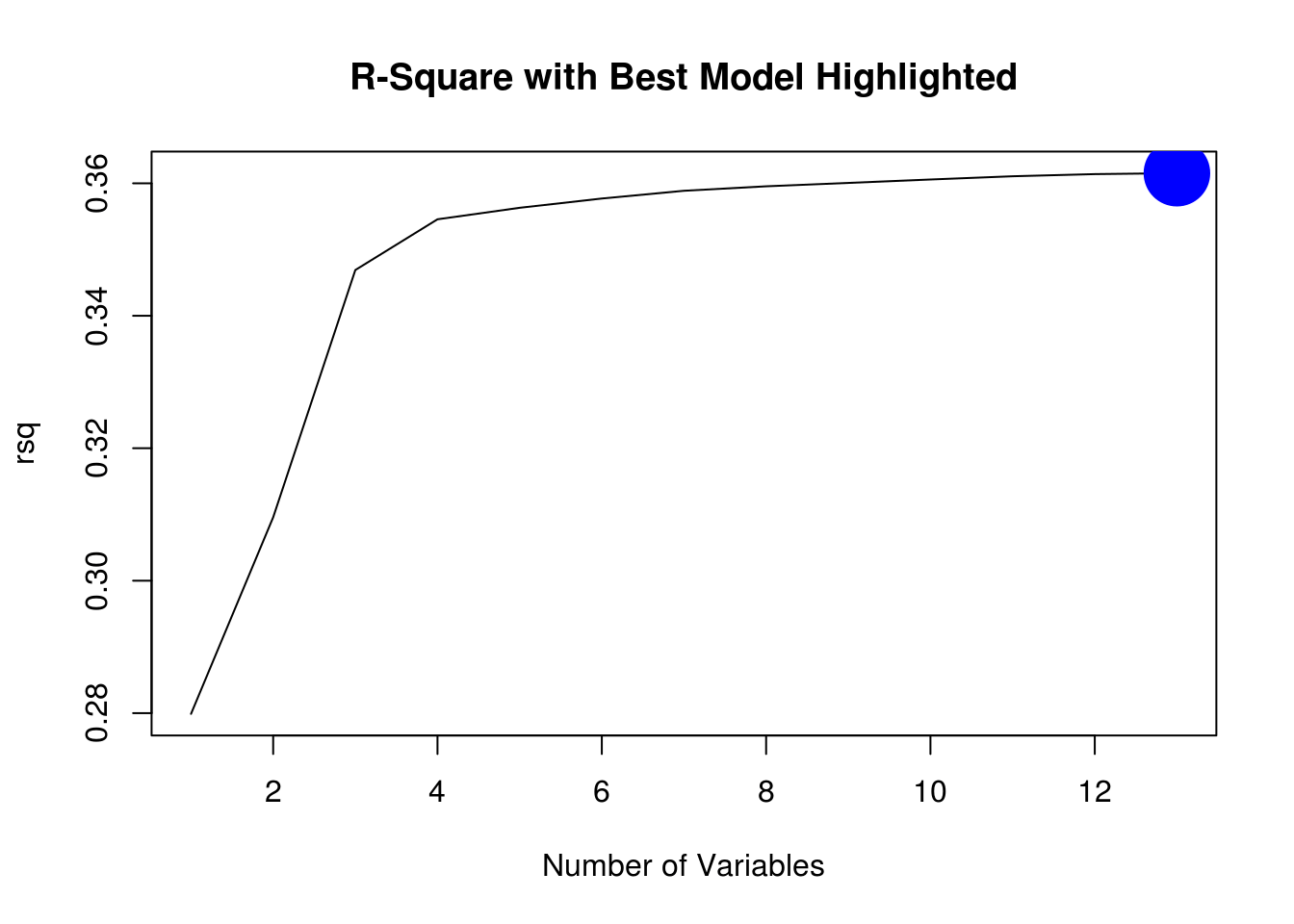``````plot(bic,type='l',main="BIC with Best Model Highlighted",xlab="Number of Variables")
points(12,(bic),col="blue",cex=7,pch=20)``````Since BIC calls for only 12 variables it is simpler than the r-square recommendation of 13. Therefore, we will fit our final model using the BIC recommendation of 12. Below is the code.

``coef(regfit.full,12)``
``````##        (Intercept)             hhiyes             whiyes
##        30.31321796         1.16940604        18.25380263
##        education.L        education^4        education^5
##         6.63847641         1.54324869        -0.77783663
##          raceblack        hispanicyes         experience
##         3.06580207        -1.33731802        -0.41883100
##            kidslt6            kids618              husby
##        -6.02251640        -0.82955827        -0.02129349
## regionnorthcentral
##         0.94042820``````

So here is our final model. This is what we would use for our test set.

Conclusion

Best subset regression provides the researcher with insights into every possible model as well as clues as to which model is at least statistically superior. This knowledge can be used for developing models for data science applications.